• 数学建模B题，刚做完，我们参考了这些材料，大家可以参考一下，有公式，不过算法没有，算法是我们自己写的，上面的算法都是由文字形式叙述的，我觉得尤其第三题，正反面都有字的复原，那个说的也特别好。
• 文章见https://blog.csdn.net/HUANGliang_/article/details/119672674 本资源包含所有《碎纸片拼接》的matlab代码以及原版论文和其他附件
• 我现在有一份文件（一张纸）的经过粉碎机粉碎后的碎片的图片，都是规则的长方形纸条，我现在要建立碎纸片拼接复原模型和算法，我该如何做，题目还说如果复原过程需要人工干预，请写出干预方式及干预的时间节点。
• [2013年国赛MATLAB创新奖B题]国防科学技术大学-碎纸片拼接复原问题.rar数学建模
• 基于旅行商模型的文字碎纸片拼接复原-浙江工业大学，好好加油，相信你的数学建模会学的更好的，好好干，数学建模要多看论文，多看看往年的题目
• 2.1问题背景： 破碎文件的拼接在司法物证复原、历史文献修复以及军事情报获取等领域都有着重要的应用。传统上，拼接复原工作需由人工完成，恢复文件...而针对本题，我们需要开发碎纸片的自动拼接技术，以提高拼接复原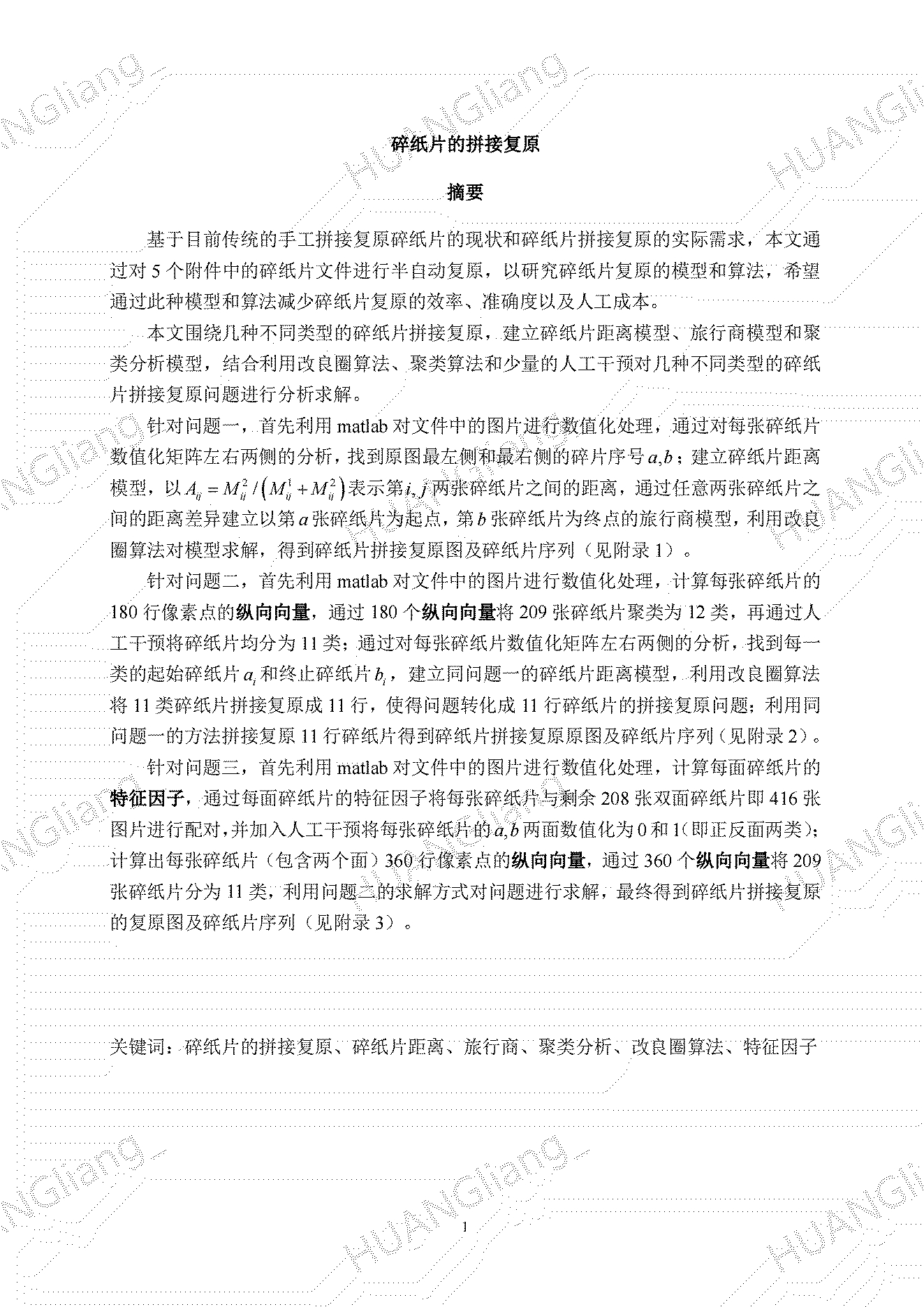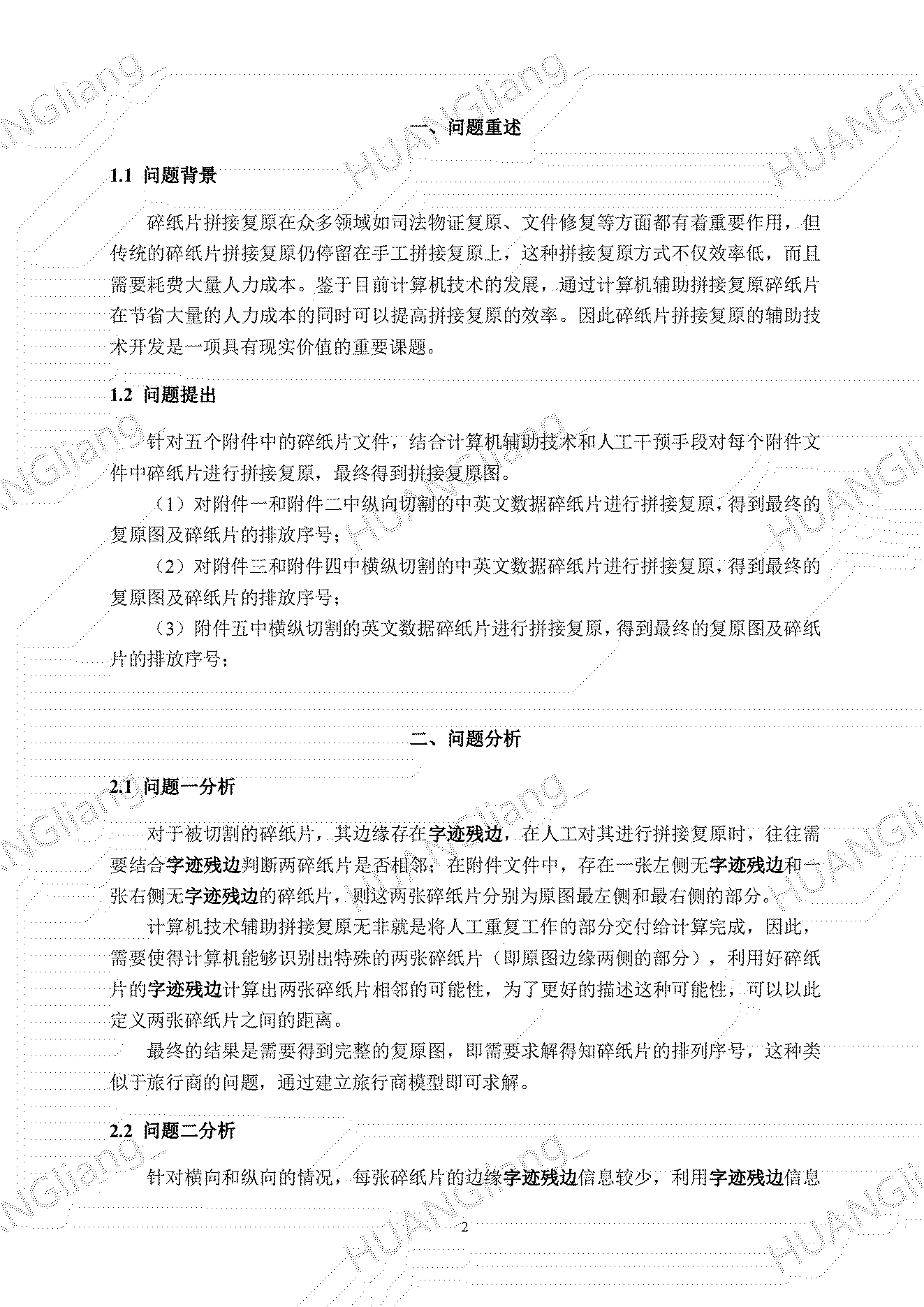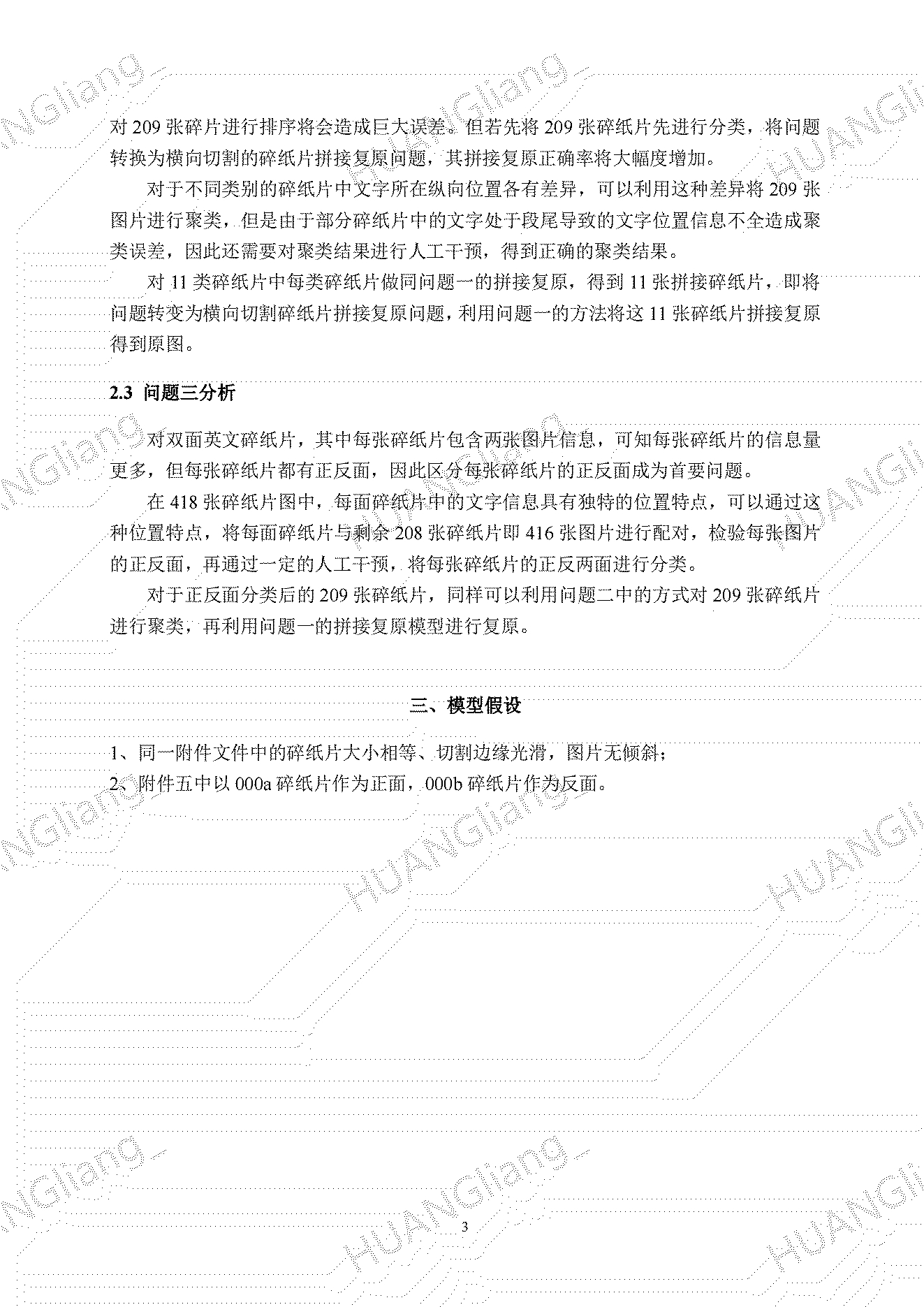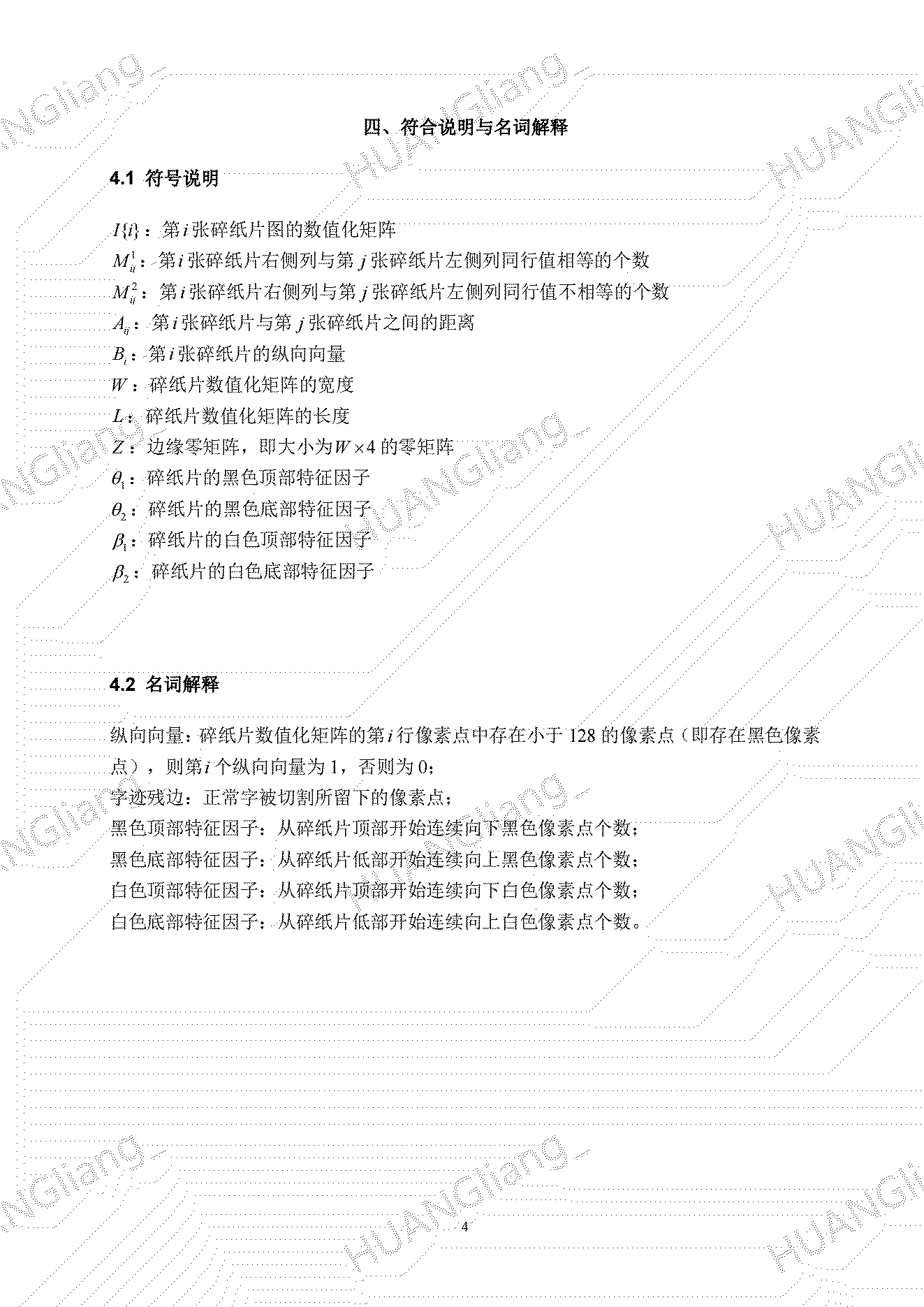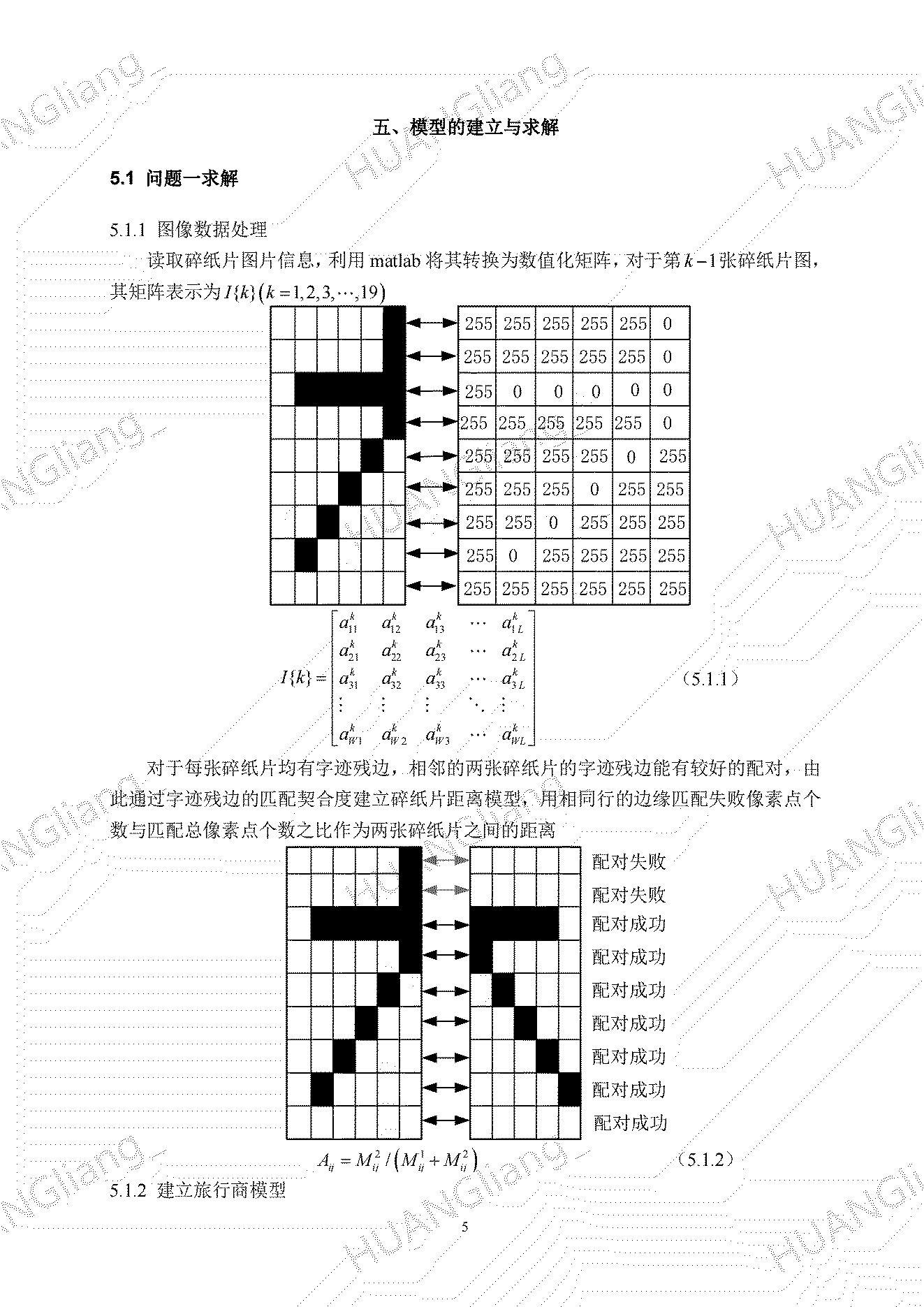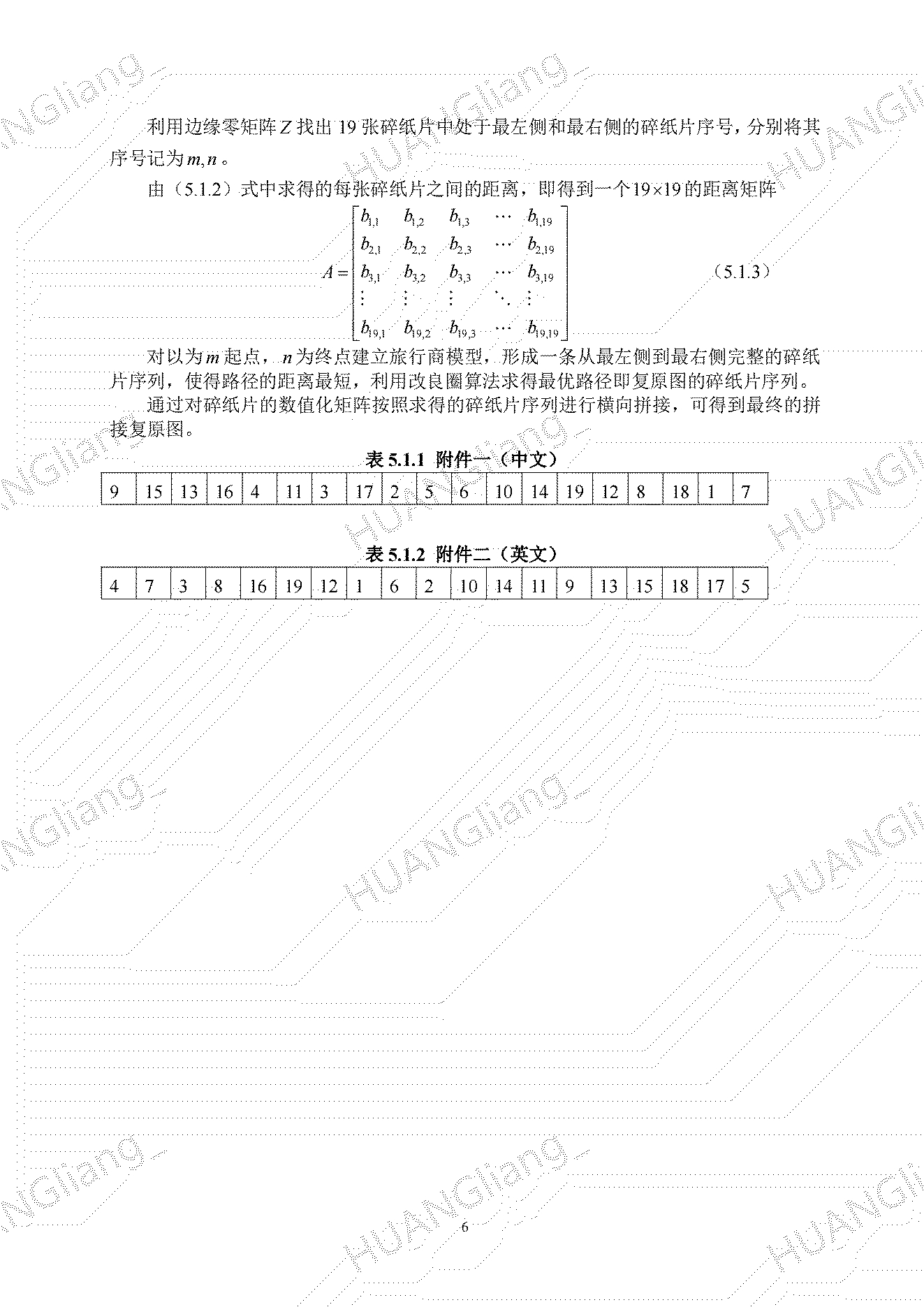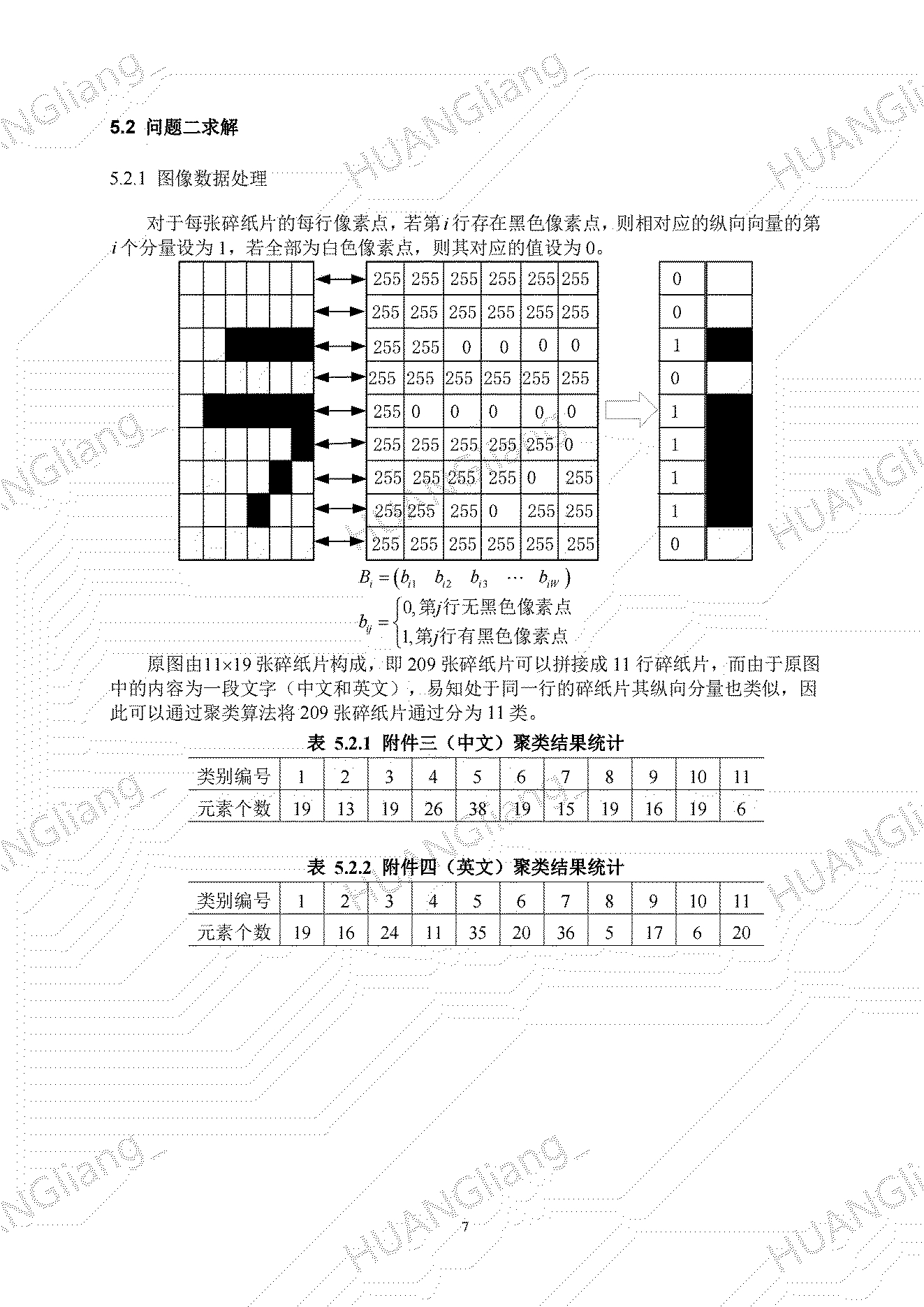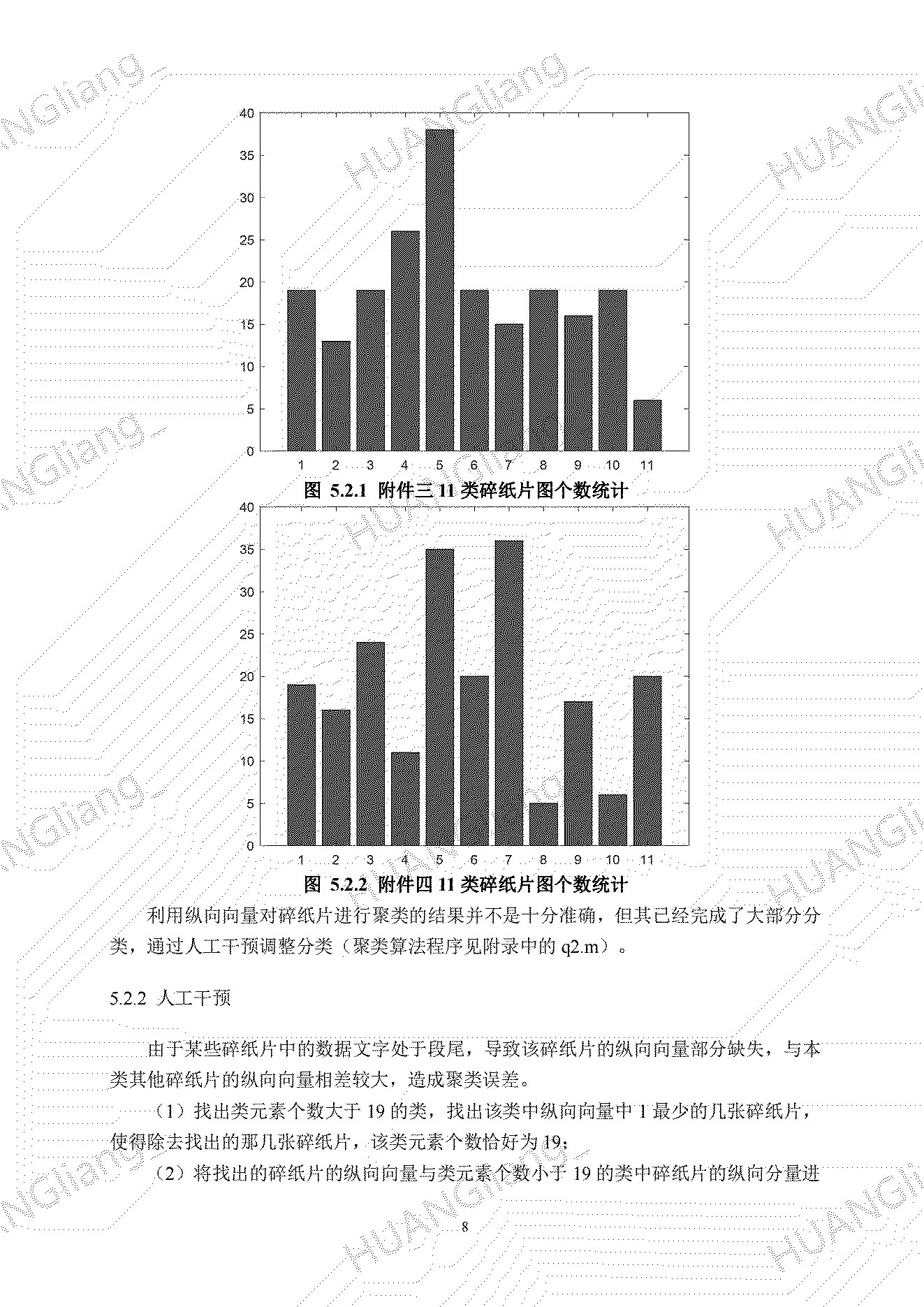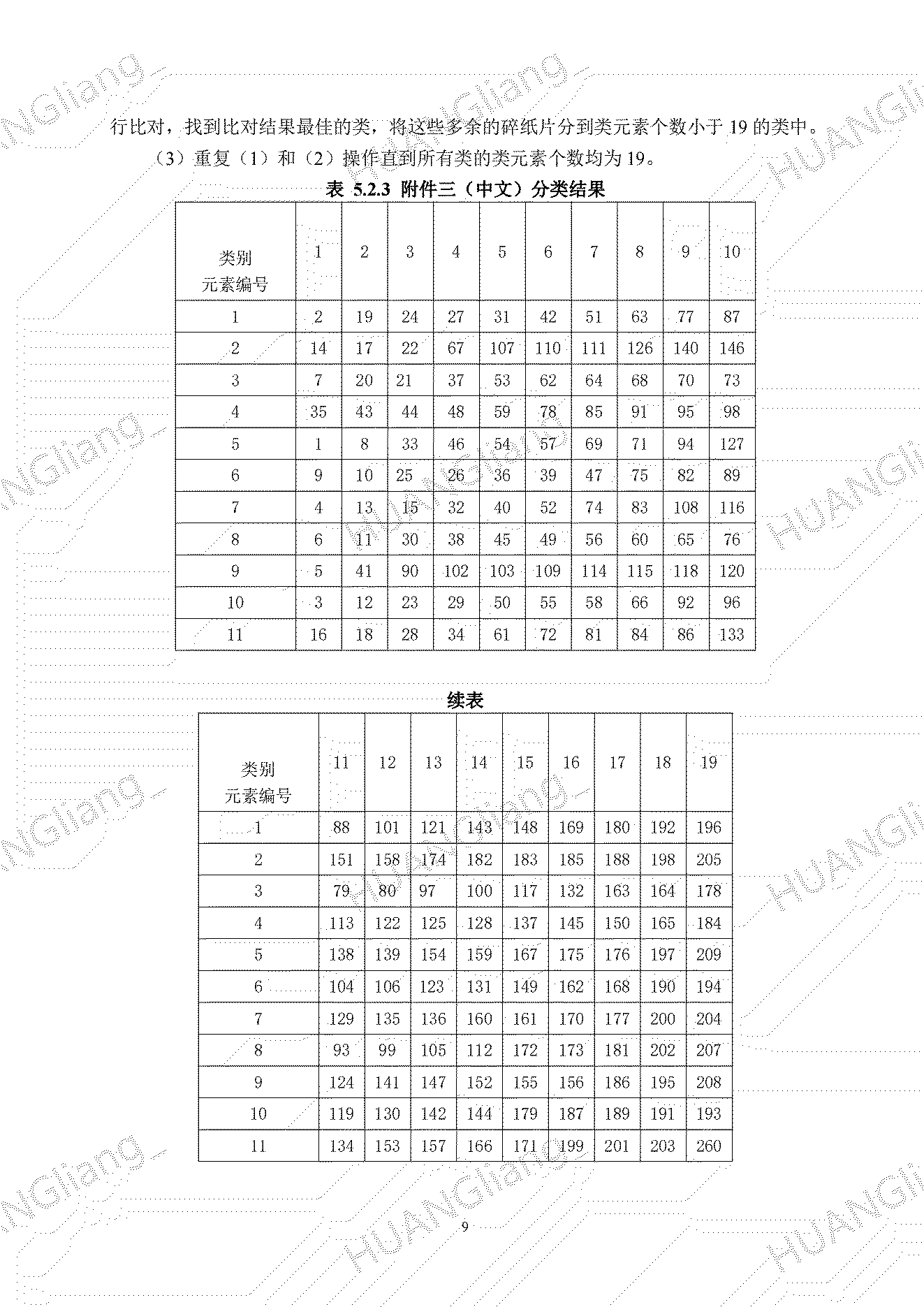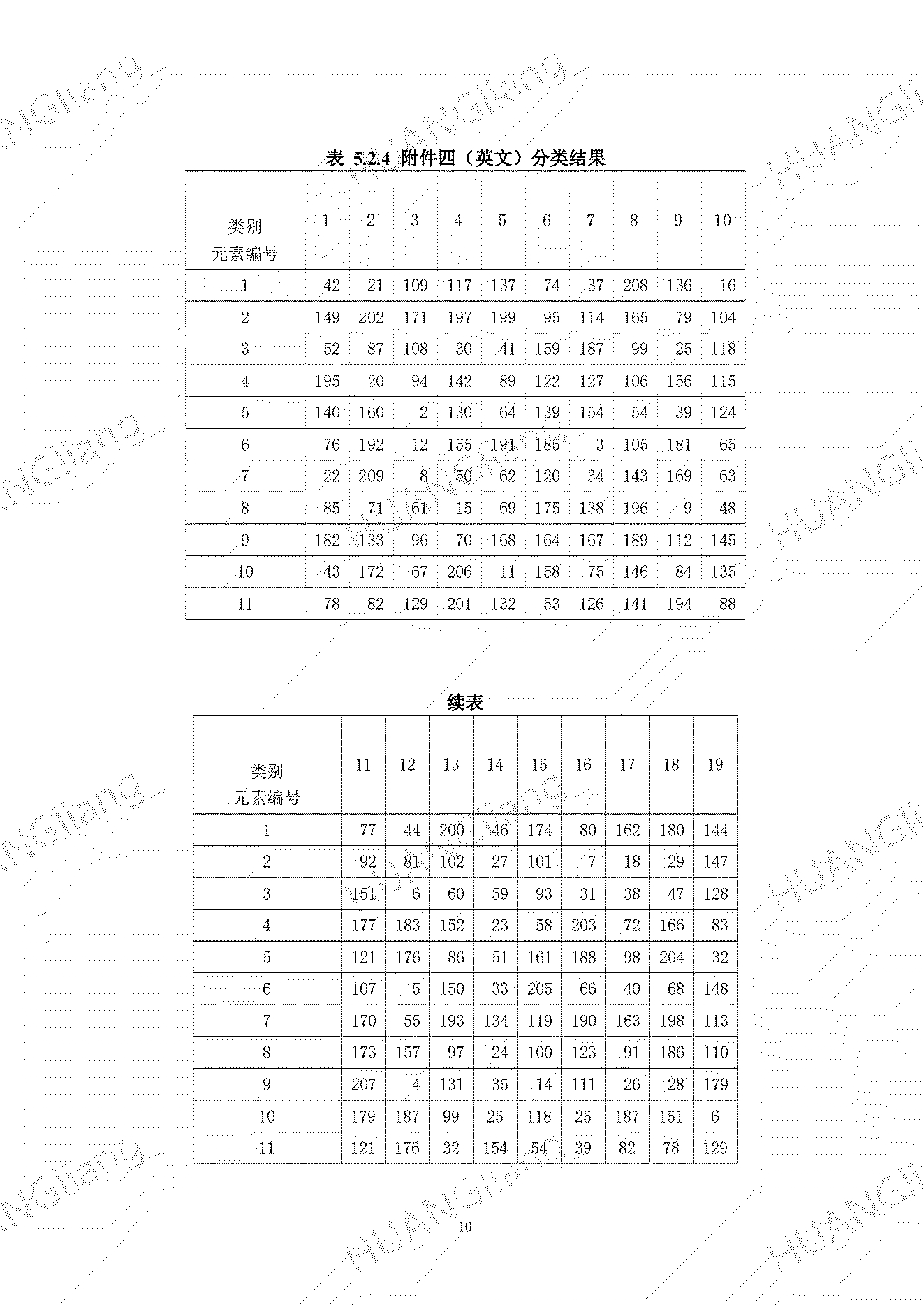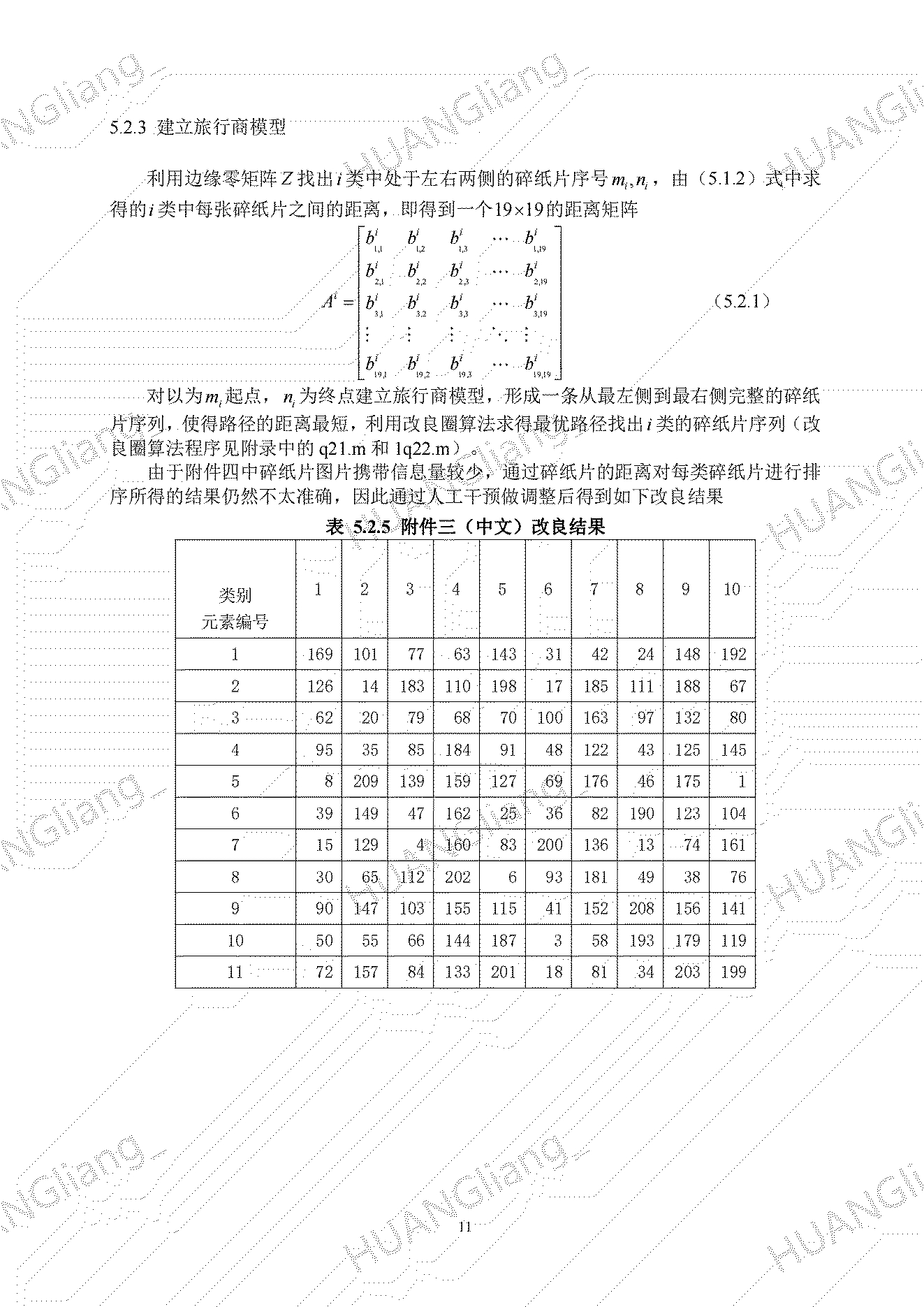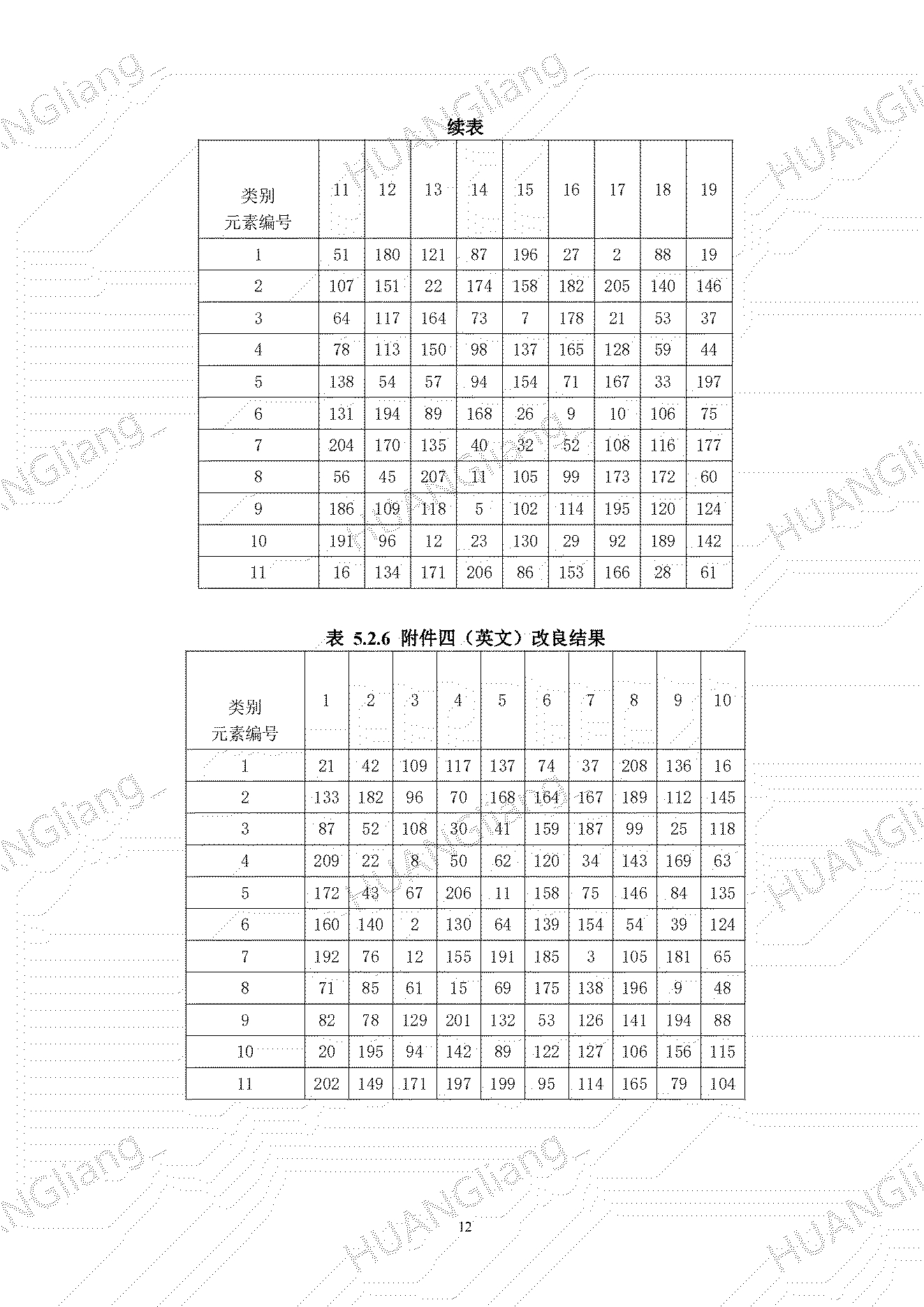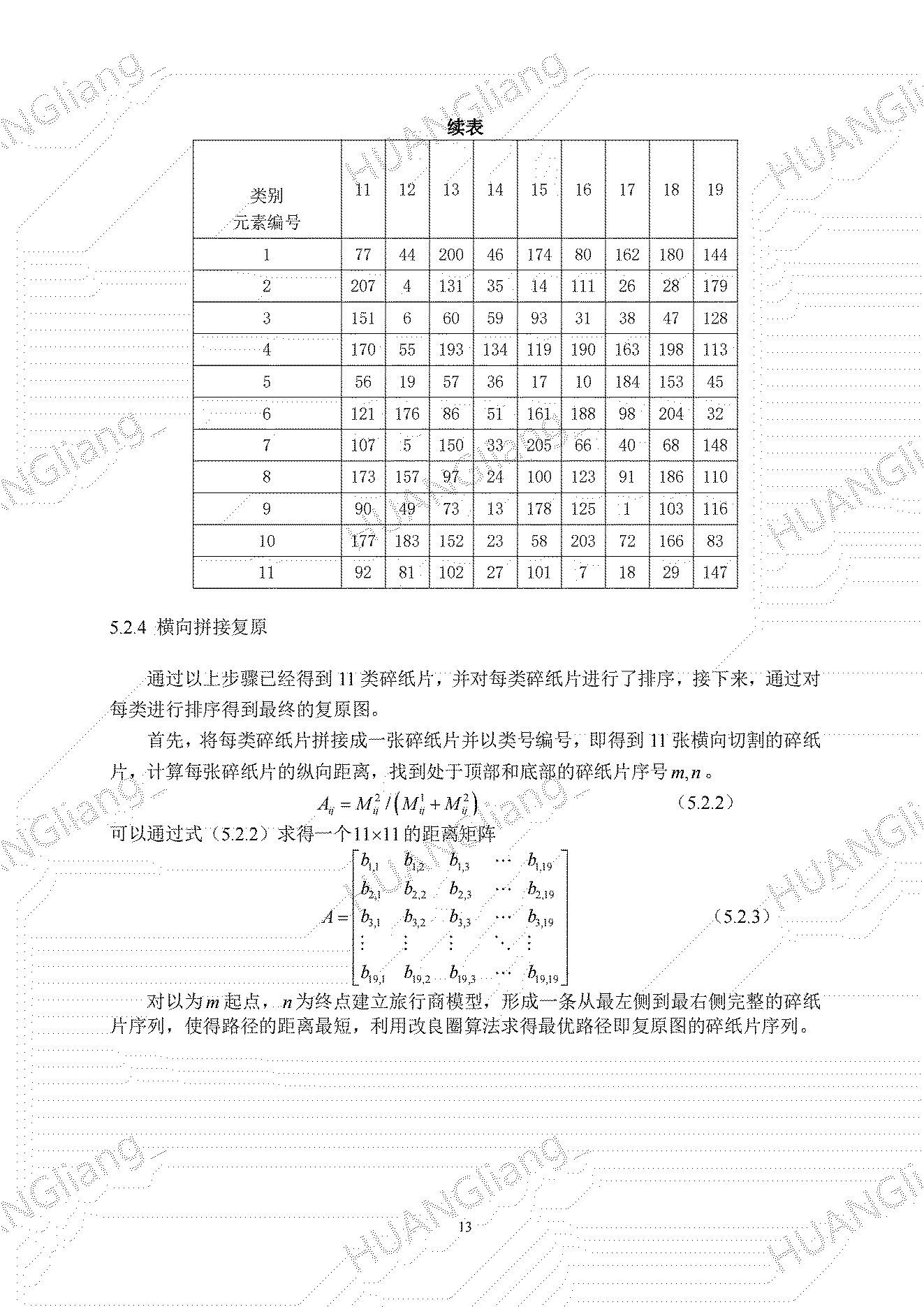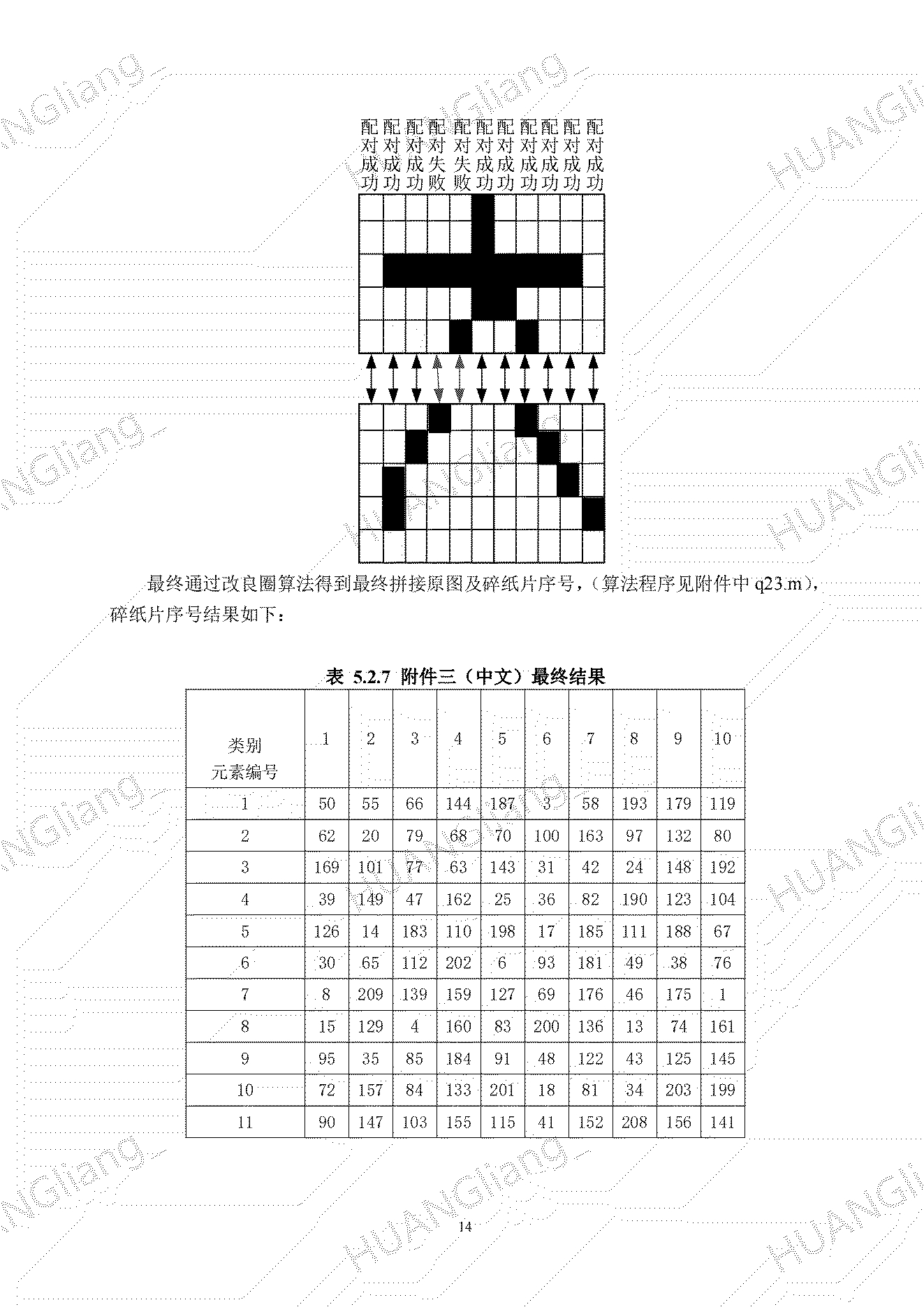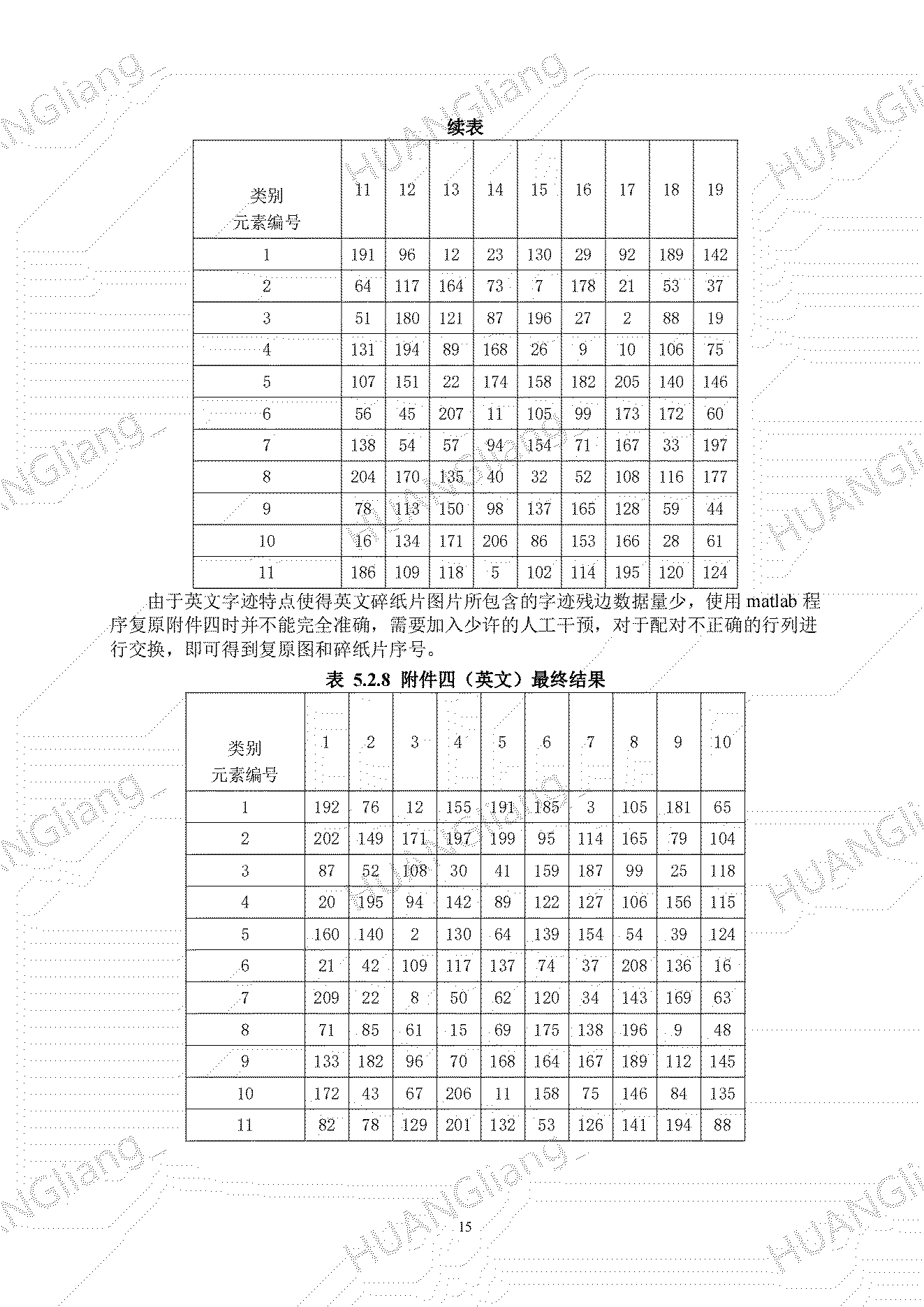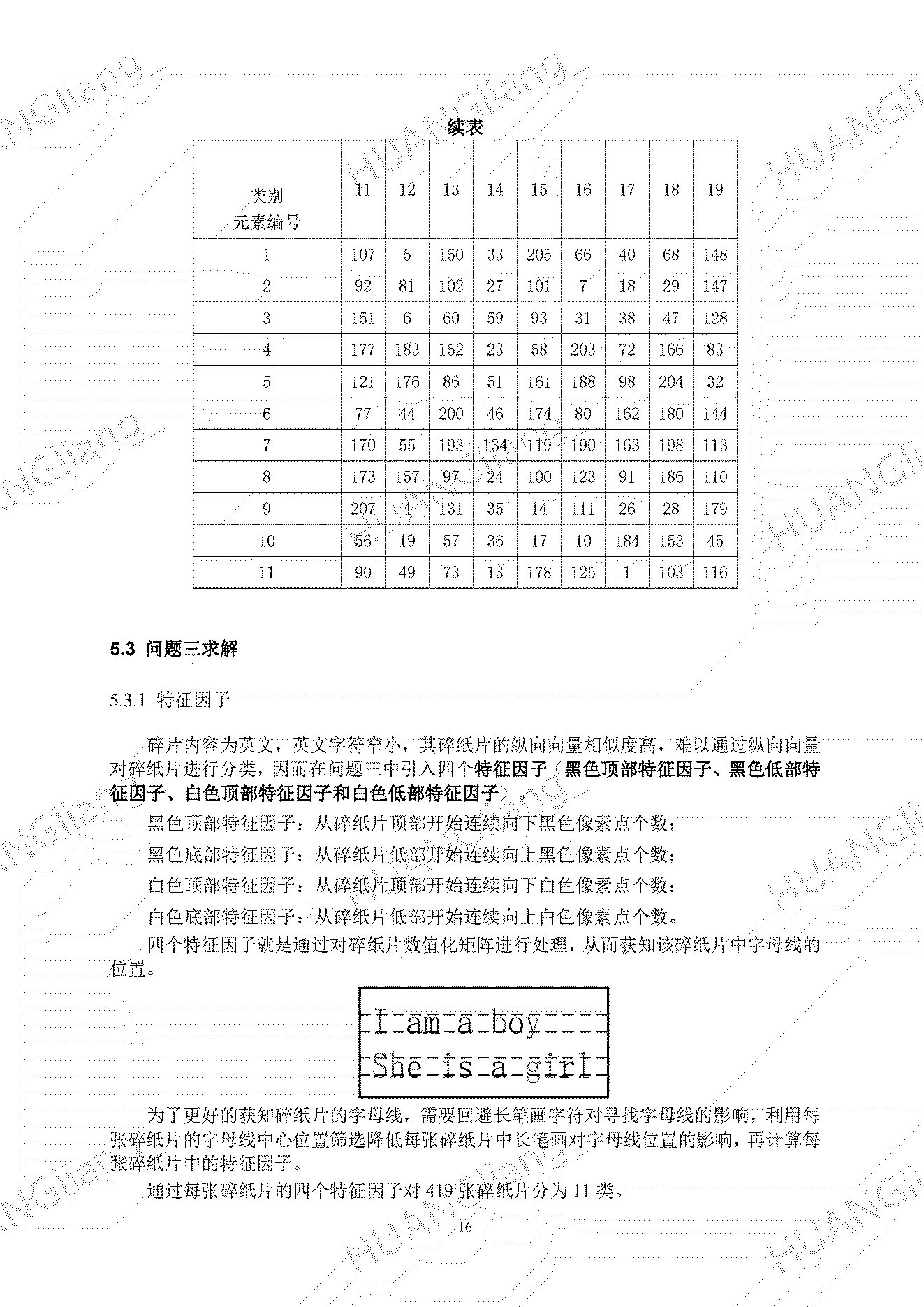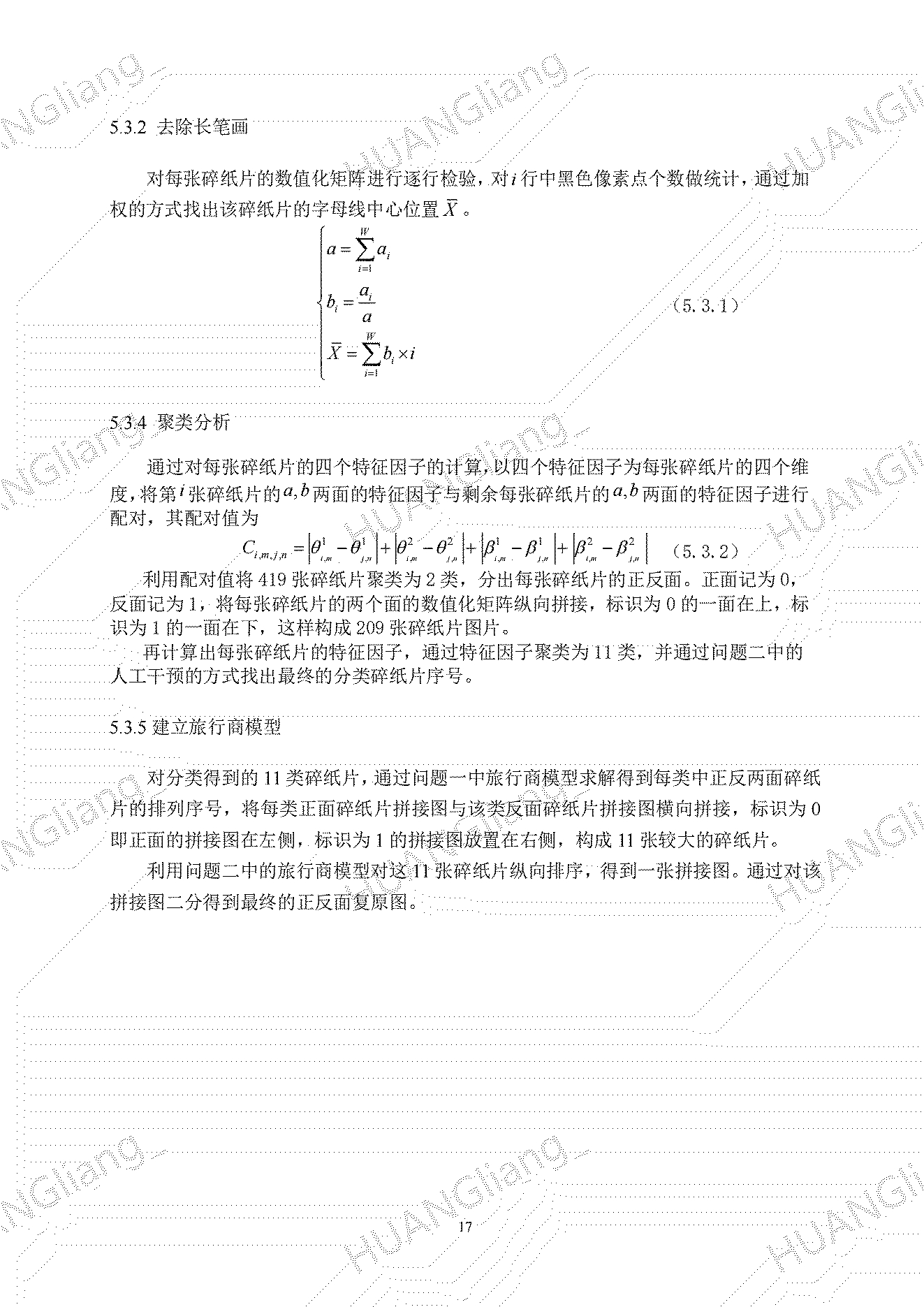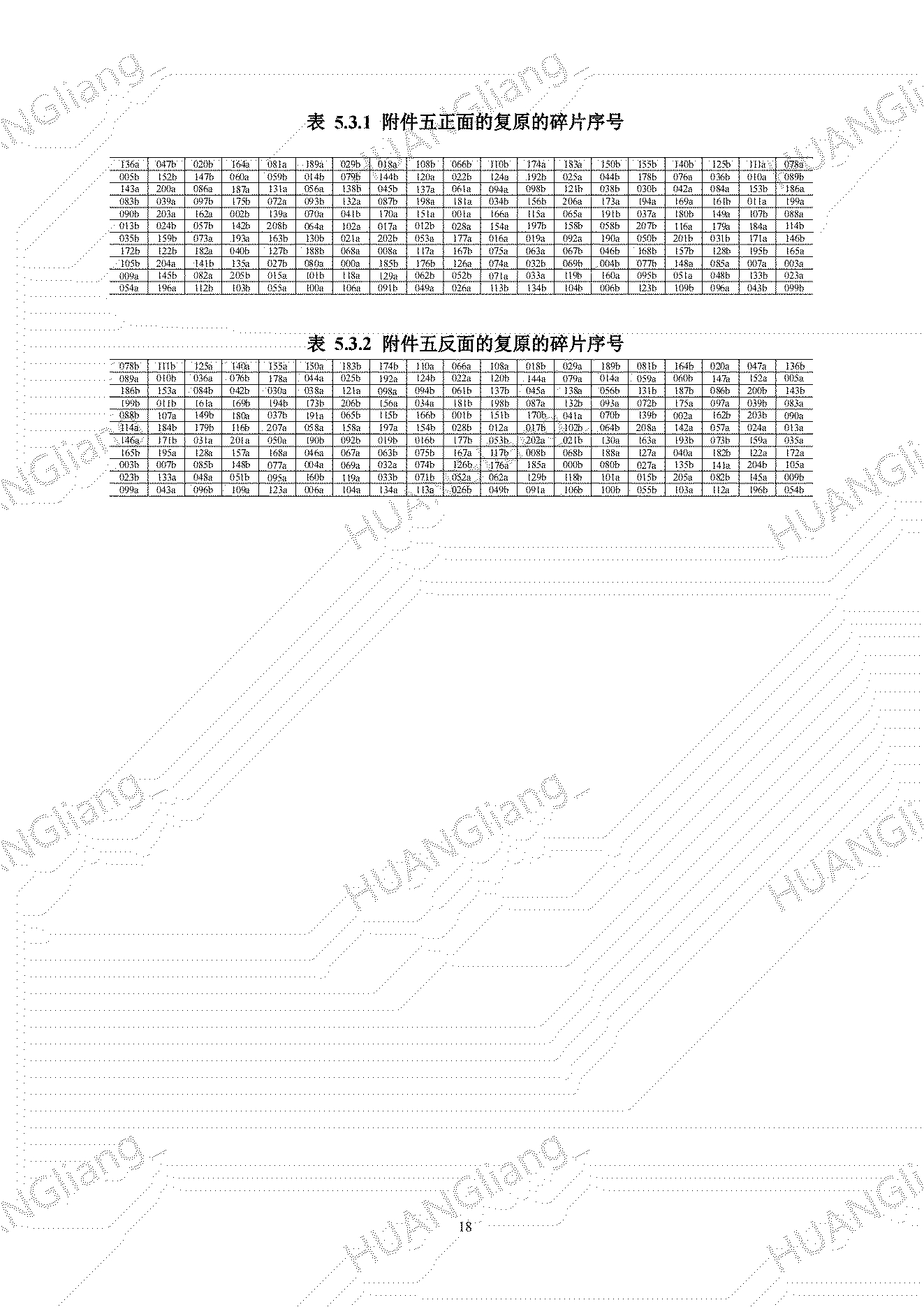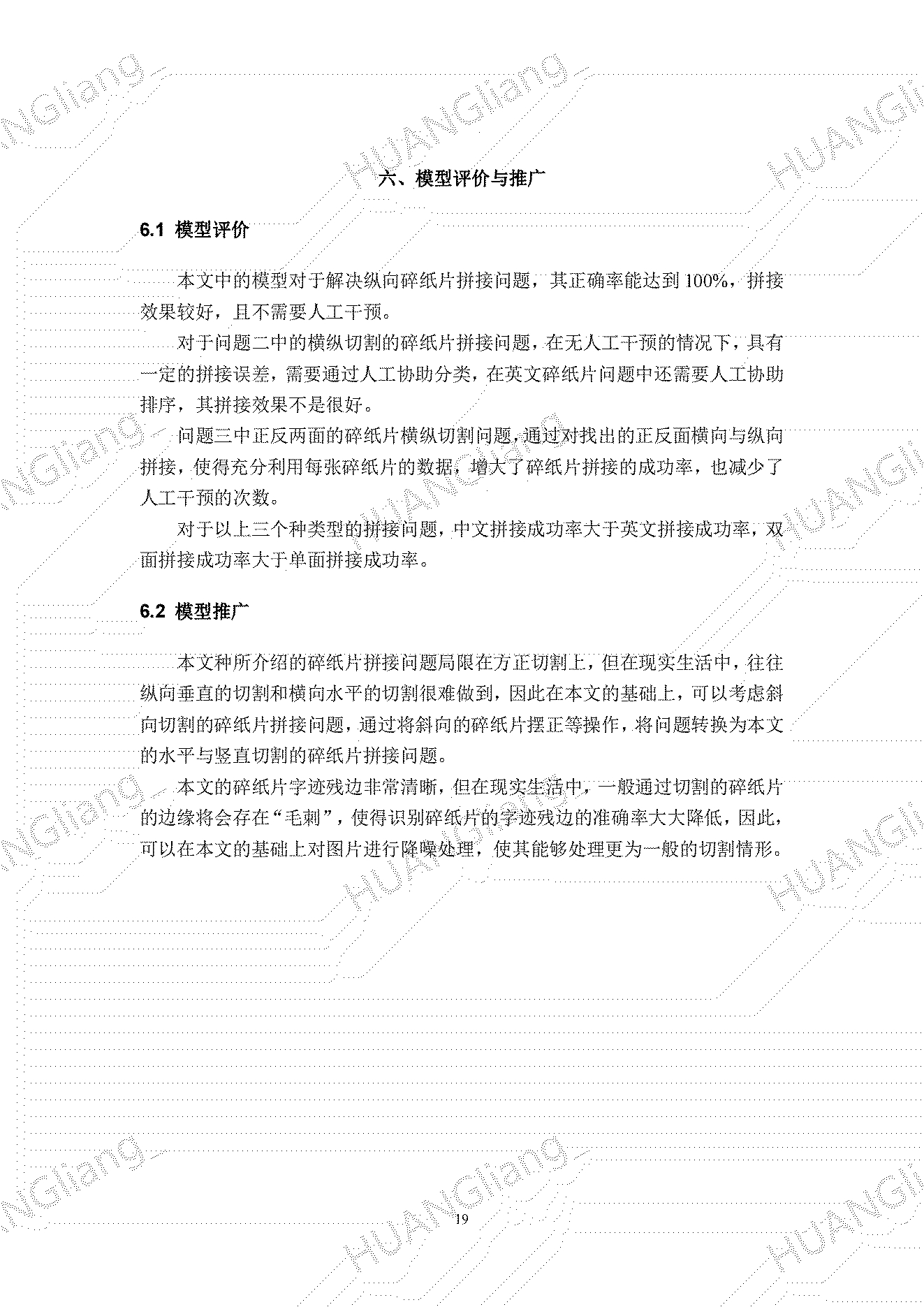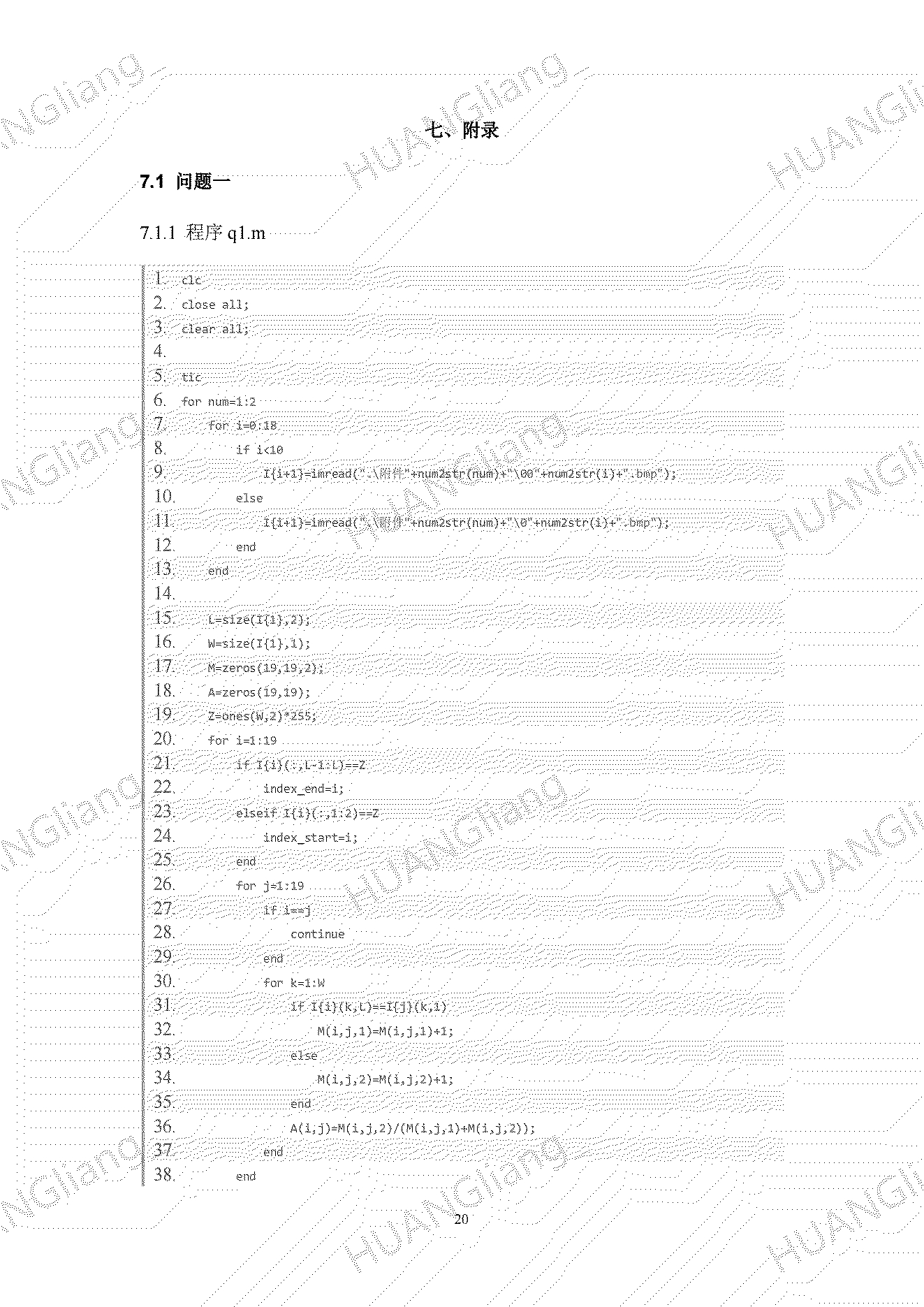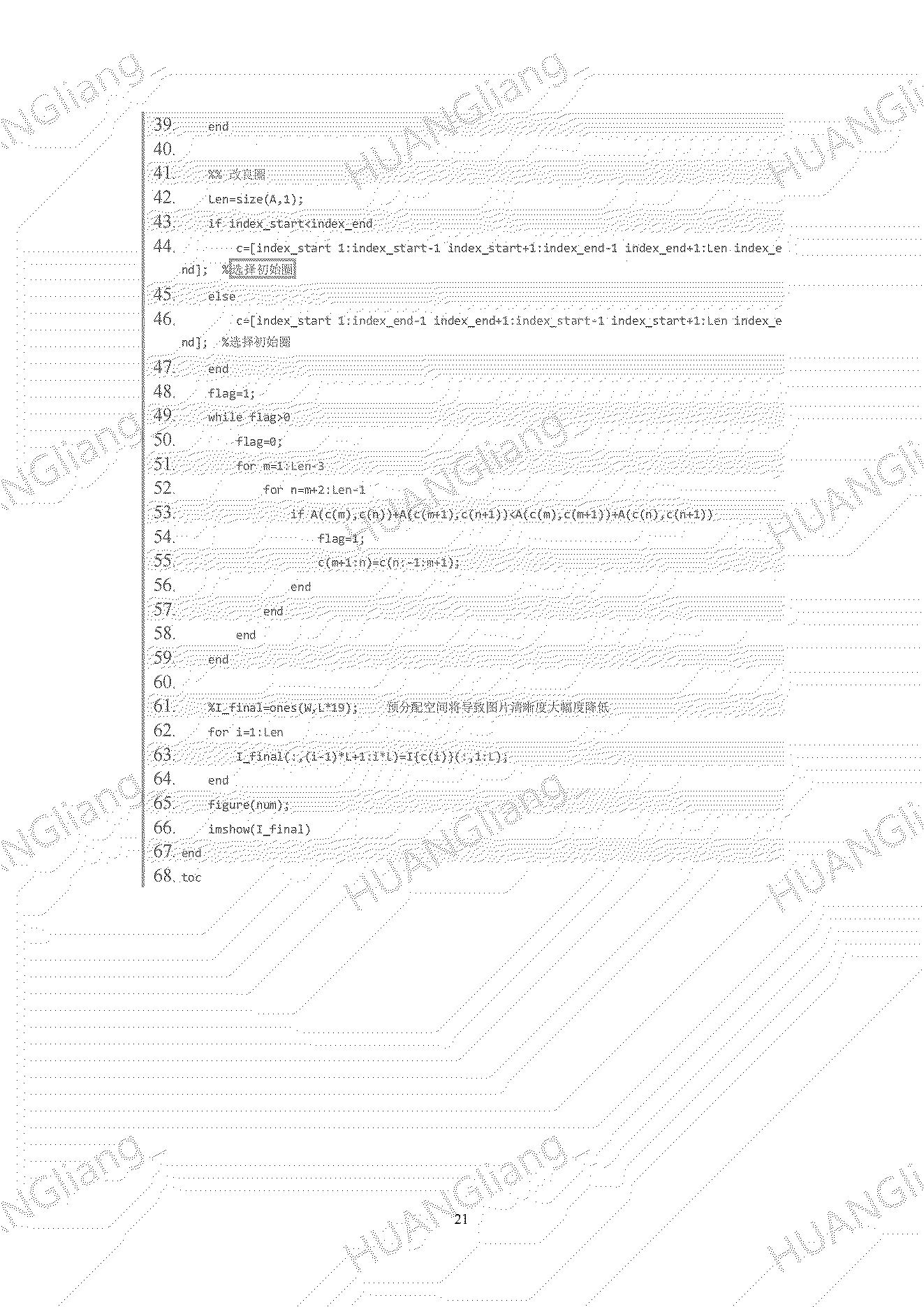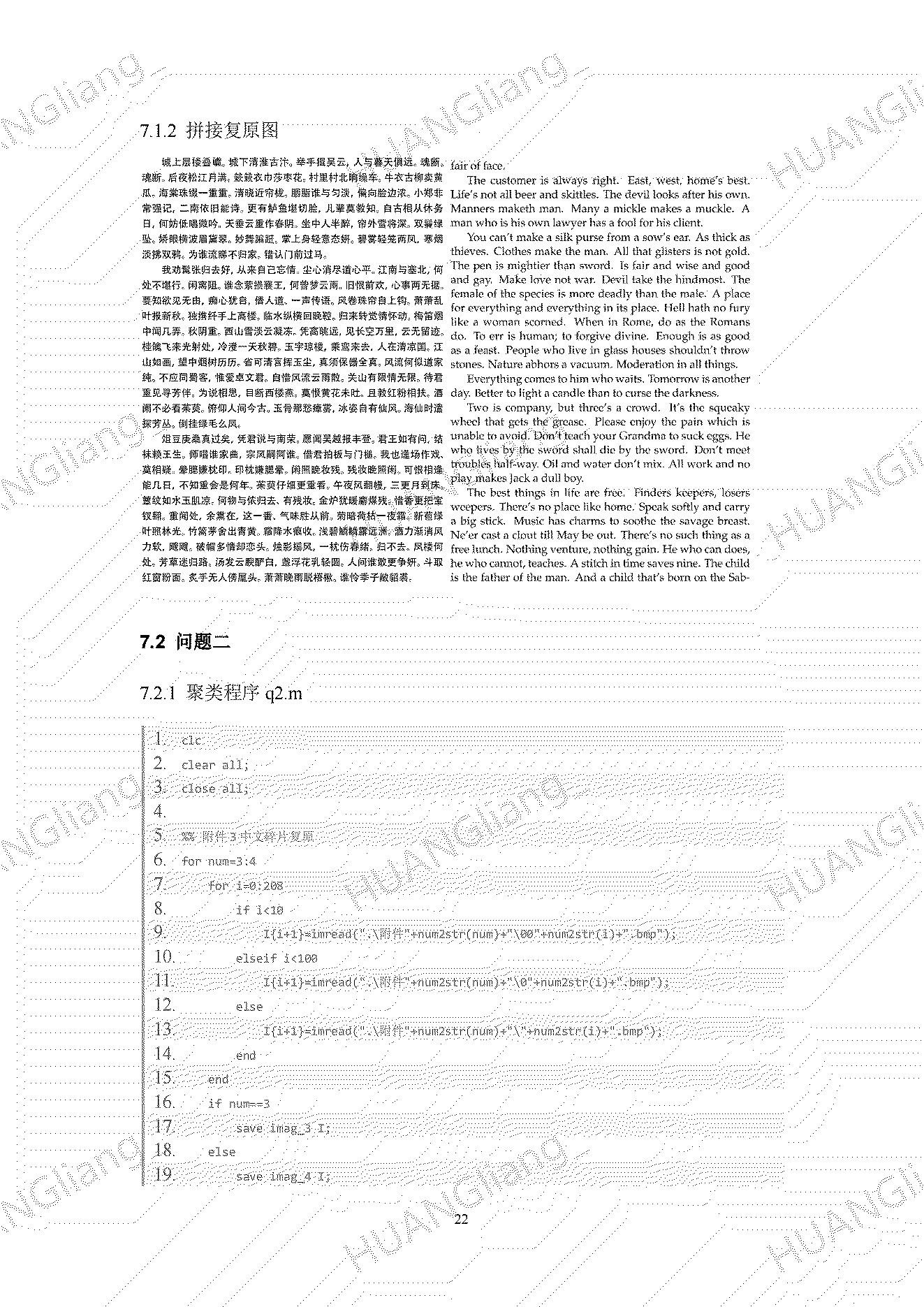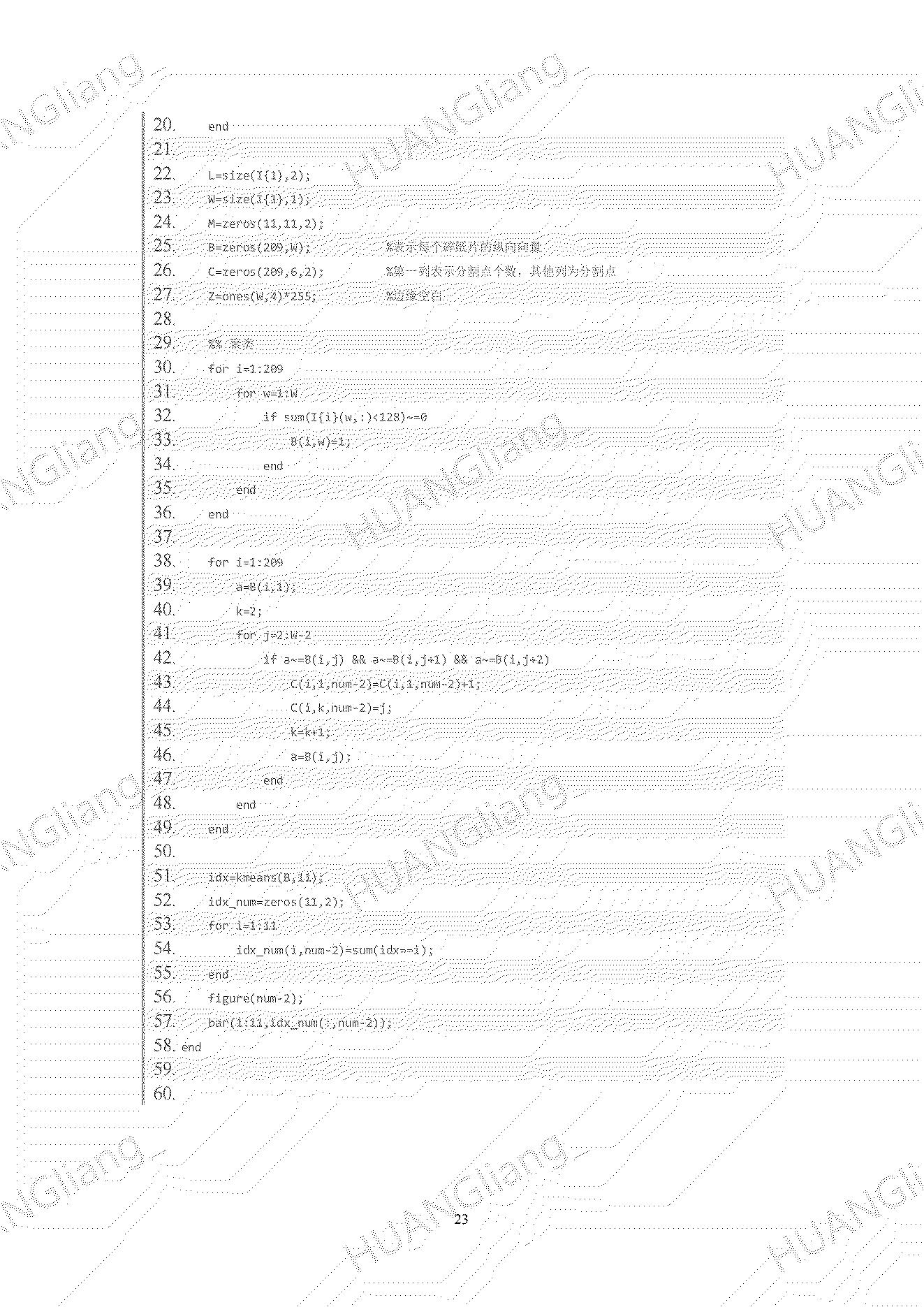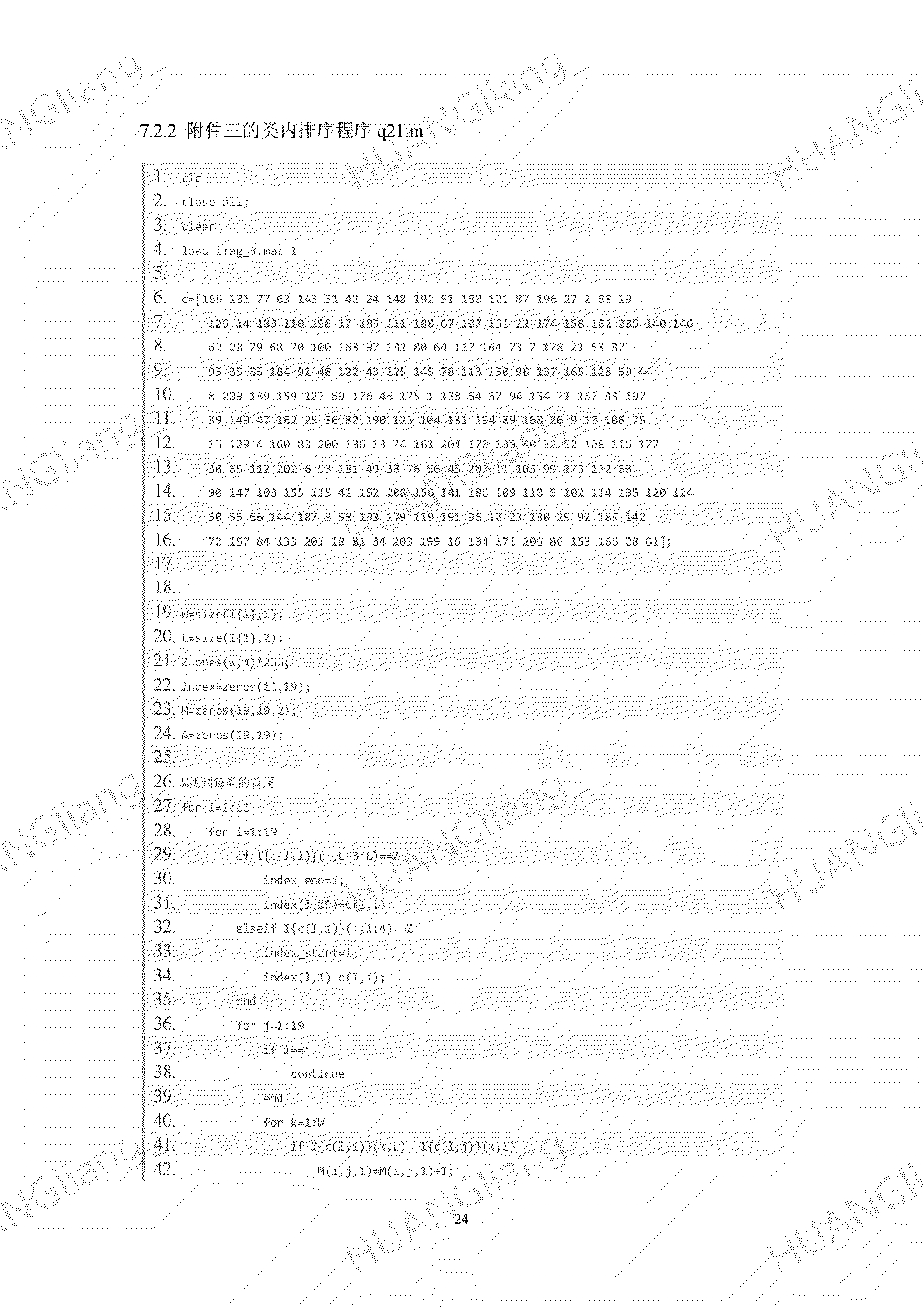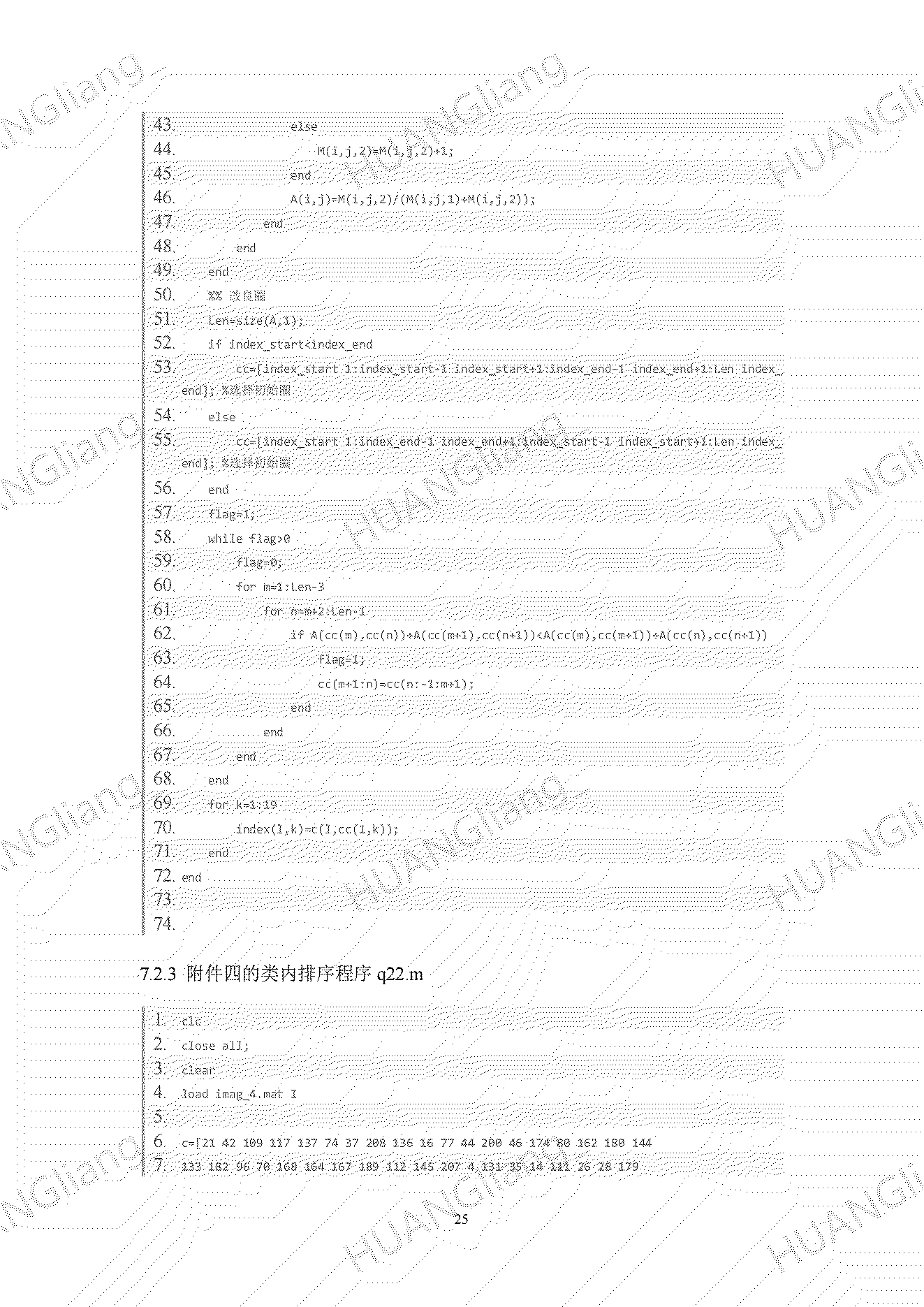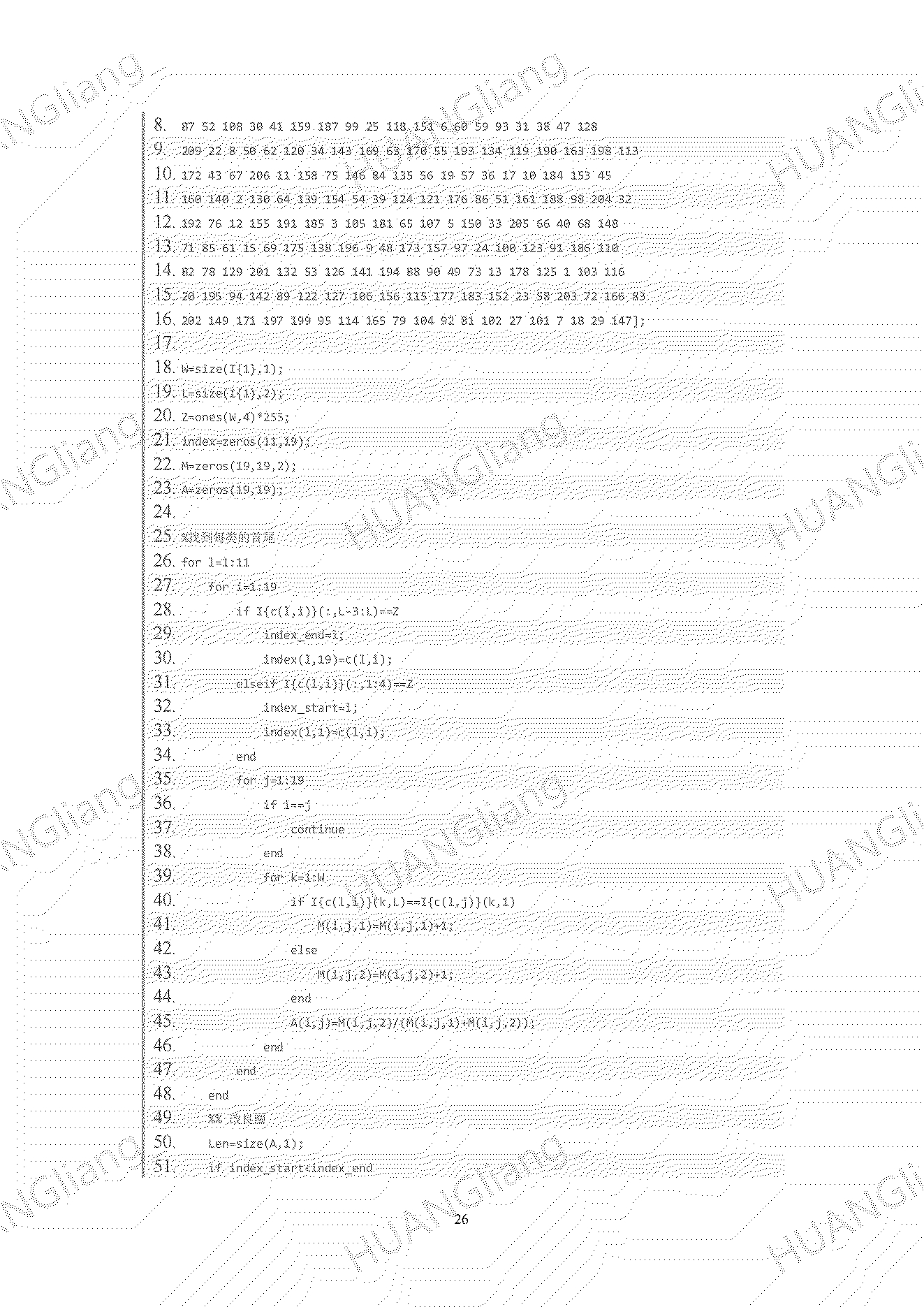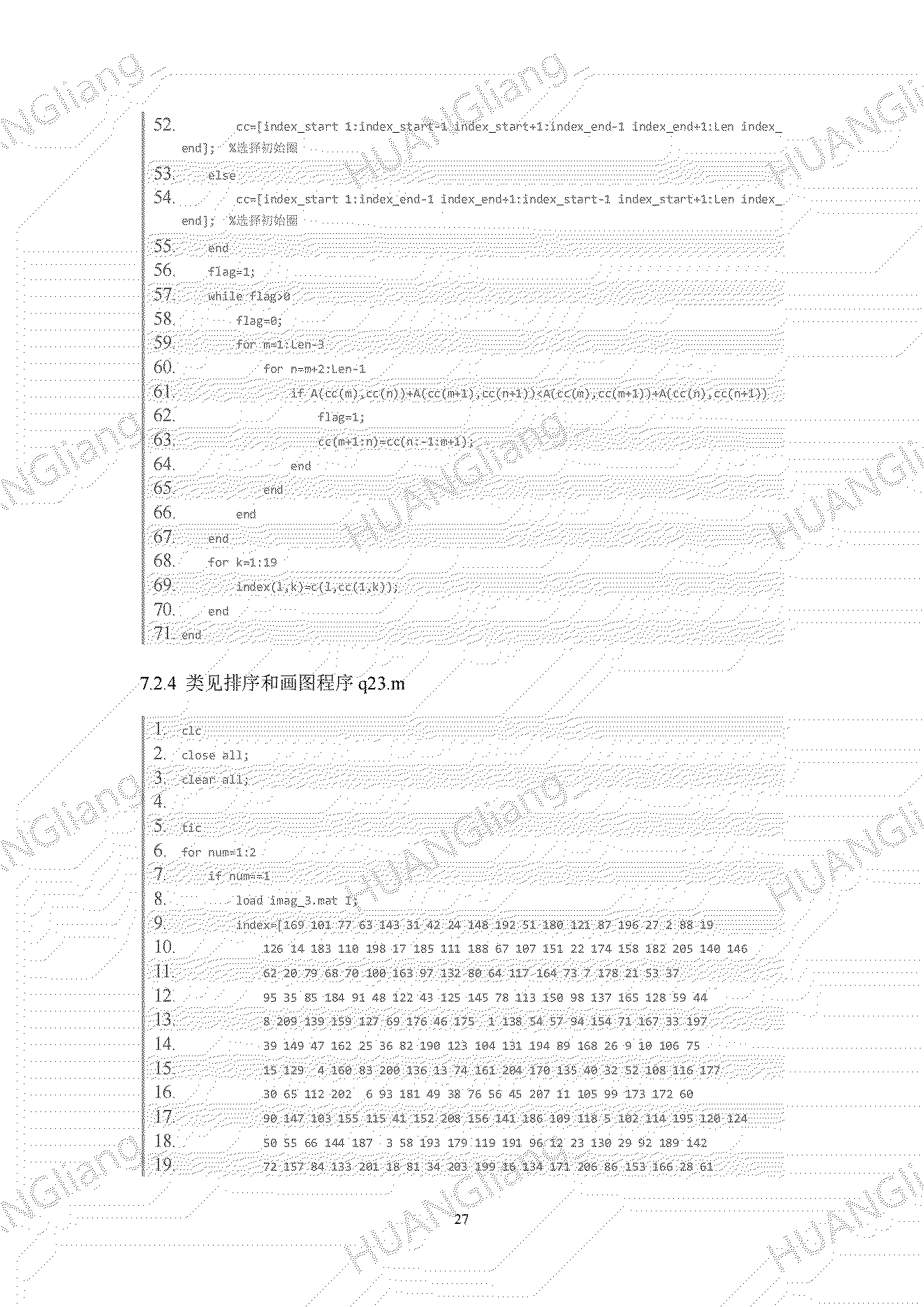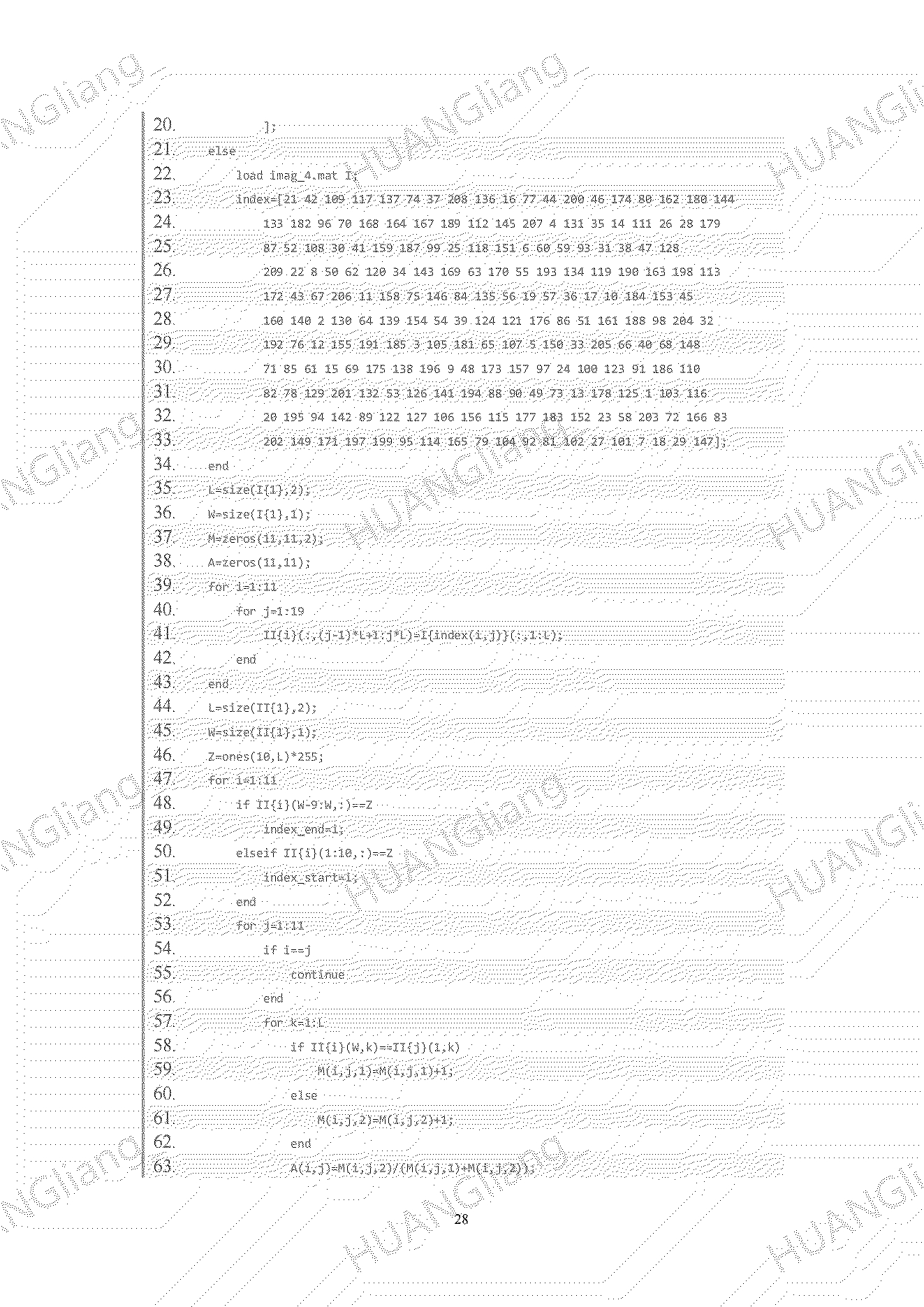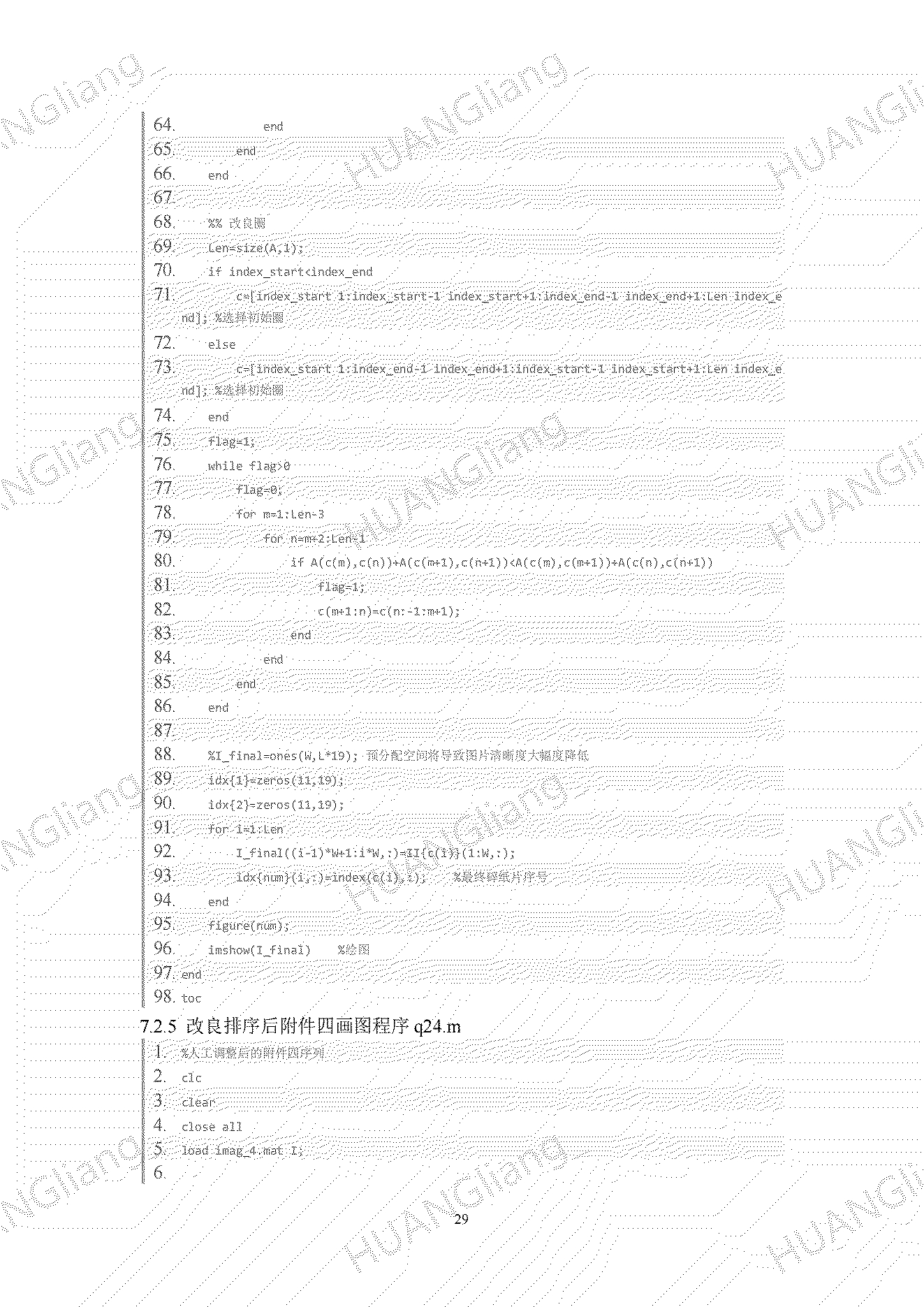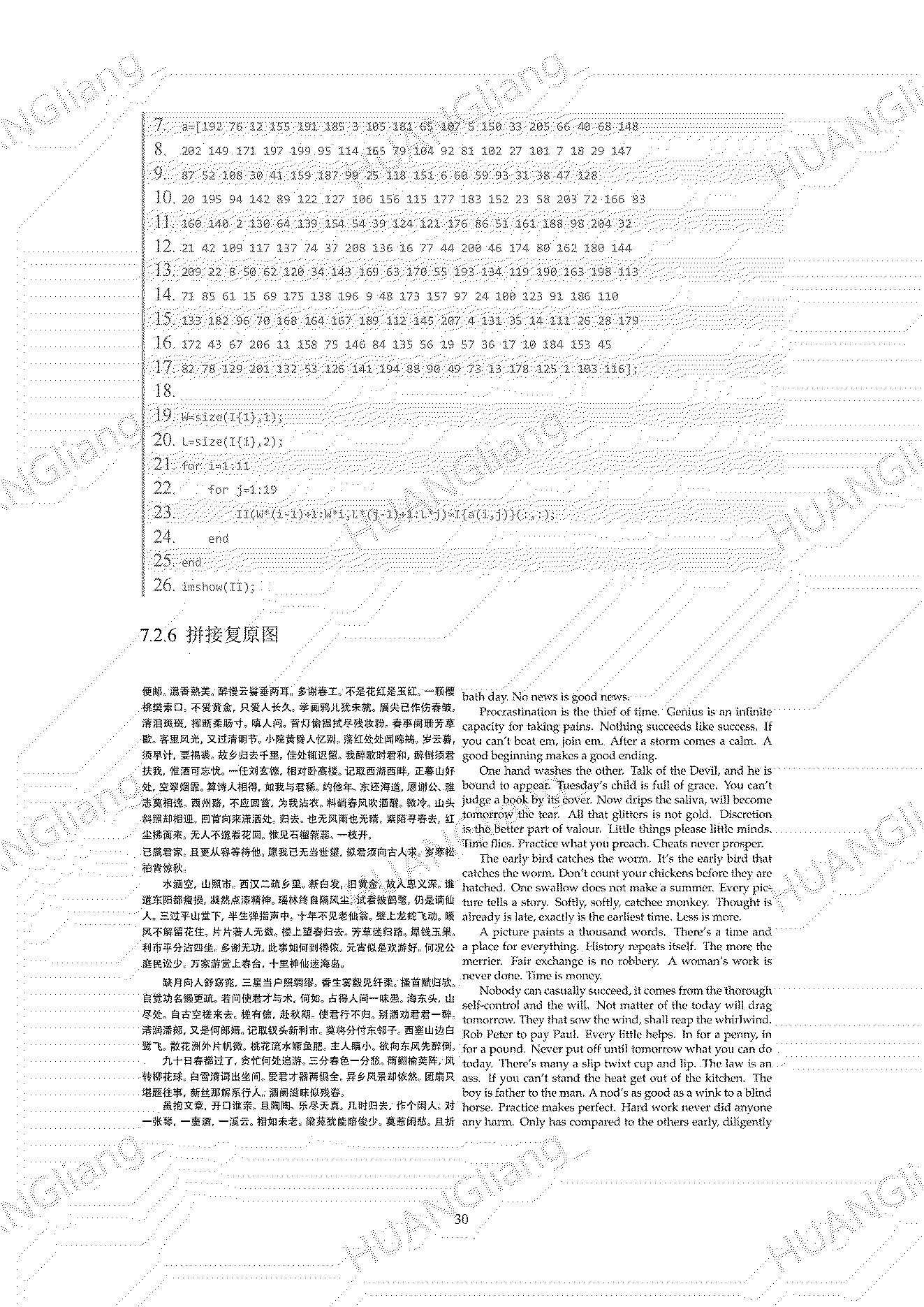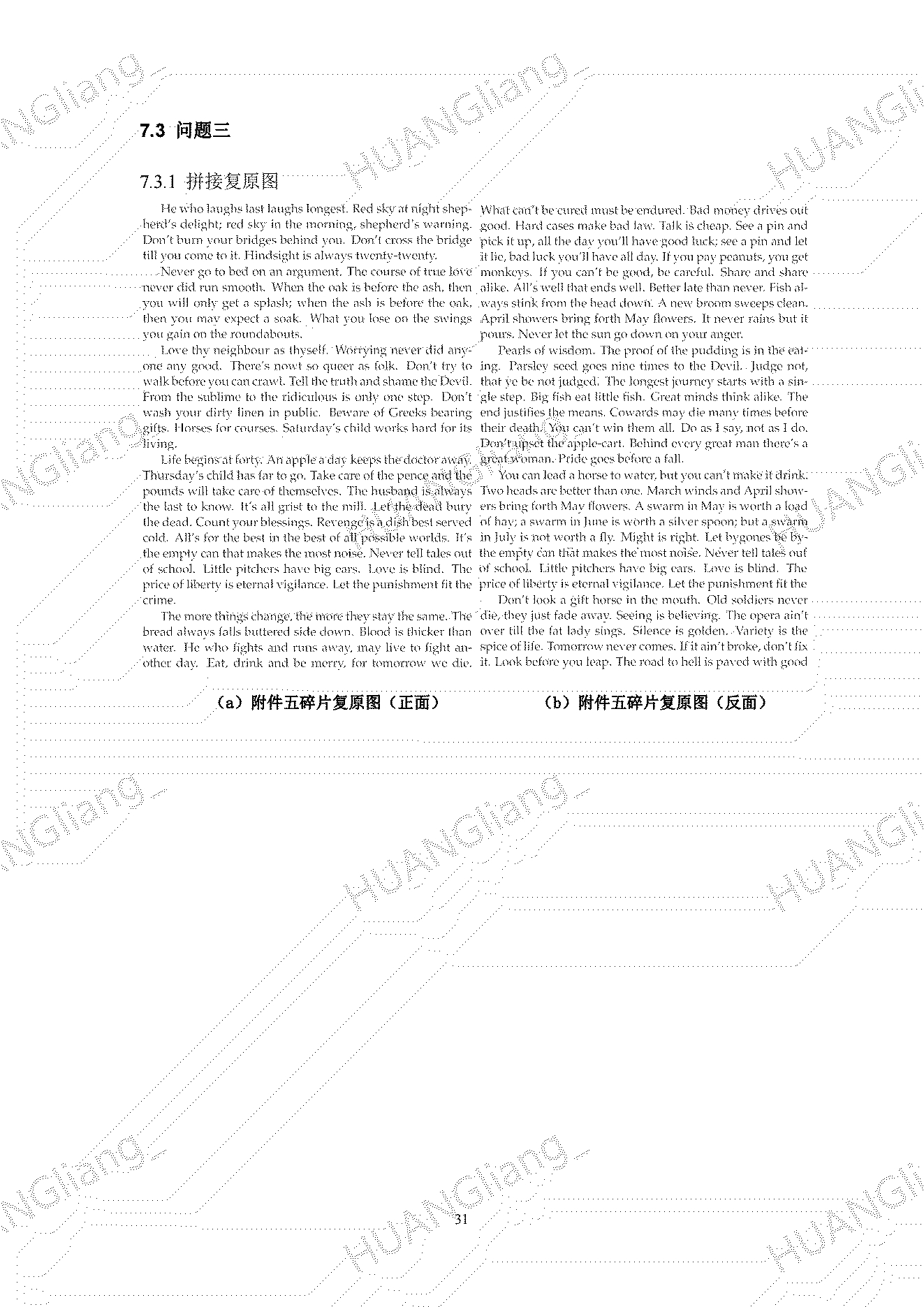仅做学习交流，代码详情见链接，原创转载请联系作者，侵权必究
资源免费下载，0积分下载
(19条消息) 碎纸片拼接复原.zip-其它文档类资源-CSDN下载
https://download.csdn.net/download/HUANGliang_/21057747
展开全文• 对于2013 的碎片拼接问题的附件一与附件二，基于旅行商算法的碎片拼接问题，寻找最优哈密顿圈 先利用matlab计算出他的边权矩阵，再用lingo规划模型，求出最优的哈密顿圈，最终可以得到正确的排序，利用人工干预，...
对于2013 的碎片拼接问题的附件一与附件二，基于旅行商算法的碎片拼接问题，寻找最优哈密顿圈
先利用matlab计算出他的边权矩阵，再用lingo规划模型，求出最优的哈密顿圈，最终可以得到正确的排序，利用人工干预，找到 初末位置，即可还原。
matlab部分代码（求边权矩阵）：
clear
close all
clc
dirname='C:\Users\Grin\Desktop\数学建模各种文档\2013年赛题\B\附件1';
files=dir([dirname,'\*.bmp']);
Num_file=numel(files);
I=cell(1,Num_file)
for k = 1:19

end

for k=1:19
J(k)=graythresh(I{k});
P{k}=im2bw(I{k},J(k));
end
for k=1:19
[le,n]=size(P{k});
left{k}=P{k}(:,1);
right{k}=P{k}(:,n);
end
for k=1:19
for n=1:19
if n==k

else
a(n,k)=sum(abs(left{n}-right{k}));
end
end
end


lingo部分代码：
model:

sets:

cities/1..19/:u;

distance,x;endsets

data:

distance=

;在此输入matlab计算的边权值矩阵

enddata

n=@size(cities);

@for(cities(i):

@sum(cities(j)|j#ne#i:x(j,i))=1;

@sum(cities(j)|j#ne#i:x(i,j))=1;

@for(cities(j)|j#gt#1#and#j#ne#i:

u(j)>=u(i)+x(i,j)

-(n-2)*(1-x(i,j))+(n-3)*x(j,i);

);

);

@for(cities(i)|i#gt#1:

u(i)<=n-1-(n-2)*x(1,i);

u(i)>=1+(n-2)*x(i,1););

end
碎片的复原代码：
circle=%输入lingo计算出的排序
sum2;
for k=1:19

circle2(k)=circle(20-k);
end
circle2

for i=1:19
begin=72*(i-1)+1;
end_f=72*i;
num=circle2(i);
all_file_new(:,begin:end_f)=I{num};
end
figure
show_file_new=all_file_new(1:1980,:);
imshow(show_file_new);


展开全文旅行商 哈密顿圈
• 碎纸片拼接复原算法，数学建模B题相关资料 比上一个便宜清晰！
• 碎纸片拼接复原 2013cumcm全国数学建模大赛B题
• 关于碎纸片复原的一篇论文，这个论文是我见过的比较好的一篇算法
• 对于碎纸机既纵切又横切的情形，请设计碎纸片拼接复原模型和算法，并针对附件3、附件4给出的中、英文各一页文件的碎片数据进行拼接复原。如果复原过程需要人工干预，请写出干预方式及干预的时间节点。复原结果表达...python
• 对于碎纸机既纵切又横切的情形，请设计碎纸片拼接复原模型和算法，并针对附件3、附件4给出的中、英文各一页文件的碎片数据进行拼接复原。如果复原过程需要人工干预，请写出干预方式及干预的时间节点。复原结果表达...有问必答 python
• 数学建模 碎纸片拼接复原 灰色关联算法 第一问： 采用灰色关联分析（完美解决~~哈哈，调试出来了） clc; clear; % 第一问代码 文件名：Qusetion1.m filename = 'E:\数学建模论文\暑假数模...

数学建模 碎纸片的拼接复原 灰色关联算法

第一问：采用灰色关联分析（完美解决~~哈哈，调试出来了）

clc; clear;
% 第一问代码 文件名：Qusetion1.m
filename = 'E:\数学建模论文\暑假数模培训\暑假作业\2013年B题碎纸片\附件1\*.bmp'
files = dir(filename);    %批量载入图像
image = cell(1, size(files, 1)) ;  % 定义一个元组

for n=1:numel(files)
end

[h,z] = size(image{1,1});            % 计算一张图片矩阵的大小(行，列)

% 只取最左和最右列进行灰色关联分析
% byz 存放 n 张图片的，最左列 (1980行, n列)
for k=1:n
byz(:, k) = image{1, k}(: , 1);      % 将第k张图片的最左边一列放入矩阵byz的第k列
byy(:, k) = image{1, k}(: , z);      % 将第k张图片的最右边一列放入矩阵byy的第k列
end

%利用页边距寻找第一张图片
for i=1:n                                     % 遍历附件1的所有图片
sum=0;
for j=1:h                                 % 遍历 图片最左列 的所有行
if byz(j,i) == 255                 % 计算255的个数
sum=sum+1;
else
break;
end
end
if sum==h                             % 如果 最左列255个数和行数一样，说明他属于第一张图片
f=i;
end
end

paixu=zeros(1,n);                       % 定义 n列向量，用来排序
index=1;
paixu(index) = f;                        % 选择第一张图片
i = f;

guanliandu = ones(n, 1);
rho = 0.45;            % 分辨系数

while index <= n - 1
cankao = byy(:, i);
byz(:, i)= nan;
t = repmat(cankao, [1, n]) - byz(:,:) ;
mmin = min(min(t));
mmax = max(max(t));
xishu = (mmin + rho .* mmax) ./ (t +rho .* mmax);
guanliandu = mean(xishu);
[gsort, ind] = sort(guanliandu, 'descend');
i = ind(1);
index = index + 1;
paixu(index) = i;
end

% 通过 系数的排序进行合成图片，在此之前，无论用什么方法，只需要求出关于关联系数的排序就好了
temp = image{ paixu(1) };
for i=2:n
temp=[temp image{ paixu(i)} ];       %将排序好的图片存放与temp
end

imshow(temp)                                    %完整显示整张纸片

方案二：采用贪心思想解决

% 第一问代码 文件名：Qusetion1.m
files = dir('E:\数学建模论文\暑假数模培训\暑假作业\2013年B题碎纸片\附件1\*.bmp');    %批量载入图像
image = cell(1, size(files, 1))   % 定义一个元组

for n=1:numel(files)
end

[h,z] = size(image{1,1});            % 计算一张图片矩阵的大小(行，列)

% 只取最左和最右列进行灰色关联分析
% byz 存放 n 张图片的，最左列 (1980行, n列)
for k=1:n
byz(:, k) = image{1, k}(: , 1);      % 将第k张图片的最左边一列放入矩阵byz的第k列
byy(:, k) = image{1, k}(: , z);      % 将第k张图片的最右边一列放入矩阵byy的第k列
end

%利用页边距寻找第一张图片
for i=1:n                                     % 遍历附件1的所有图片
sum=0;
for j=1:h                                 % 遍历 图片最左列 的所有行
if byz(j,i) == 255                 % 计算255的个数
sum=sum+1;
else
break;
end
end
if sum==h                             % 如果 最左列255个数和行数一样，说明他属于第一张图片
f=i;
end
end

paixu=zeros(1,n);                       % 定义 n列向量，用来排序
index=1;
paixu(index) = f;                        % 选择第一张图片

%依据连续性模型需找匹配点
for i=2:n                                   % n - 1 张图片
max=0;
for j=1:n                               % n 张图片
sumofbp = 0;                   % 选取非白色点进行匹配，匹配过程中，非白色点的总数记为sumofb
xishu = 0;                         % 用来计算 灰色关联系数 (最大匹配率)
for k = 2 : h-1                  % 一张图片(2, h-1)行
if byy(k, paixu(index)) ~= 255       %最右一列(第k行, 当前一张图片0~18) != 255 ==> 非白色
if byz(k,j) < 255 || byz(k-1,j) < 255 || byz(k+1, j) < 255     % 最左一列(k附近三行, j张图片) => 非白色
xishu = xishu+1;
else
xishu = xishu;
end;
end
end
if xishu > max
max = xishu;
signal = j;
end
end
index=index+1;
paixu(index)=signal;                       %存放排好序的图片序号
end

temp = image{ paixu(1) };

for i=2:n
temp=[temp image{ paixu(i)}];       %将排序好的图片存放与temp
end

imshow(temp)                                    %完整显示整张纸片（中英文都可以复原）
第二问：（等待实现）.....

posted @
2017-08-20 18:40
douzujun 阅读(
...) 评论(
...)
编辑
收藏


展开全文• 国赛数学建模关于碎纸片拼接复原的程序有用到的赞一个吧
• ## 碎纸片的拼接复原

万次阅读 多人点赞 2017-03-03 14:28:06
本文旨在建立模型，利用计算机编程加少量的人工干预实现碎纸片拼接复原。 针对问题1，由于对任意一个字符来讲，笔画是连续的，所以对于一般情况，即使文字被切开，两边的像素还是有联系的，是呈现离散性渐变的。...

摘  要
对重要文件的拼接复原，传统上都由人工完成，拼接准确率虽然高但是效率很低。本文旨在建立模型，利用计算机编程加少量的人工干预实现碎纸片的拼接复原。
针对问题1，由于对任意一个字符来讲，笔画是连续的，所以对于一般情况，即使文字被切开，两边的像素还是有联系的，是呈现离散性渐变的。针对此特征，建立了文字连续性模型来求解该问题。而又因为被切开的两边碎片边缘灰度是具有高相关性的，所以，又建立了向量相关模型来求解进行图片拼接。用两种模型分别求解，都得到了对附件一和附件二的正确拼接结果。
针对问题2，可以采用第一问的模型，先进行全局搜索，找出每个碎片最相似的右侧邻近碎片，拼接出每一行的图片，再利用向量相关性进行横向拼接，得到拼接结果。但是，由于纸片同时被横向与纵向切割，碎片小，数量多，碎片之间的信息量不够，容易造成误判。所以建立向量投影分类模型，首先将可能处于同一行的碎片用模糊C均值(FCM)聚类方法分到同一类。对于汉字或英文的缺行碎片（碎片中只有一行或者两行字），利用掩码补充模型将投影中缺失的行用掩码补齐，然后再放入分类。分类完成后，将每一类中的碎片进行横向排序拼接。每一类横向拼接完成后，从而拼接成完整图片。对于不能正确拼接的部分，采取了多种人工干预的策略，最终得到正确的拼接结果。
针对问题3，由于附件中的碎片分为正反两面，所以可以建立组合匹配模型将碎片首先将正反面碎片的灰度矩阵上下拼接，每张碎片的拼接方案有两种（灰度矩阵a放在左右镜像处理后的b上或灰度矩阵b放在左右镜像处理后的a上）。拼接过后，边缘灰度向量信息会增加为原来的两倍，这时利用问题二中的全局搜索模型用向量相关性的方法进行匹配拼接。拼接出所有行后，将每一行的下半部分矩阵切割放在上半部分的右边，又可增加横向拼接时的边缘信息，然后各行再次利用向量相关性的方法进行横向拼接，最终得到结果图，但是仍然存在较多的碎片不能正确匹配，需要人工干预。
由于碎片在切割时的随机性，使得机器自动拼接存在一定的难度，因此，采取恰当的人工干预的措施，是保证正确拼接的必要手段。

关键词：文字连续性  匹配  FCM聚类方法 掩码补充模型  向量投影分类模型

一．问题背景及重述
破碎文件的拼接在司法物证复原、历史文献修复以及军事情报获取等领域都有着重要的应用。传统上，拼接复原工作需由人工完成，准确率较高，但效率很低。特别是当碎片数量巨大，人工拼接很难在短时间内完成任务。但如果借助计算机技术，开发出碎纸片的自动拼接技术，提高拼接复原效率将会大大提高。
问题1：给定的来自同一页印刷文字文件的碎纸机破碎纸片（仅纵切），建立碎纸片拼接复原模型和算法，并且拼接复原附件1和附件2给出的中、英文各一页文件的碎片数据。
问题2： 对于碎纸机既纵切又横切的情形，设计碎纸片拼接复原模型和算法，并针对附件3和附件4给出的中、英文各一页文件的碎片数据进行拼接复原。
问题3：从现实情形出发，还可能有双面打印文件的碎纸片拼接复原问题需要解决。附件5给出了一页英文印刷文字双面打印文件的碎片数据。设计相应的碎纸片拼接复原模型与算法，并就附件5的碎片数据给出拼接复原结果。
二．模型假设
1、不计碎纸片边缘的磨损，没有边缘像素损耗；
2、假设纸张垂直放入碎纸机即每张小碎纸片都是规则矩形；
3、图片中的文字像素没有任何断点；
4、图像上没有任何噪声或污点造成像素干扰。
三．变量说明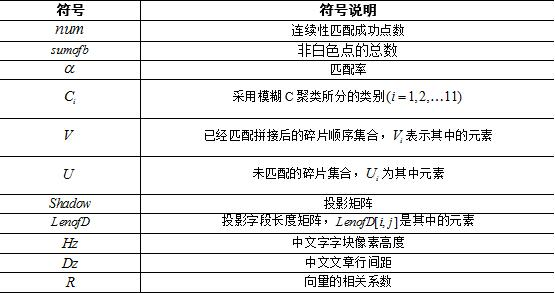四．模型准备与问题分析
针对题目中附件所给的图片信息，我们学习准备了图像的处理方法、模式匹配方法和图像拼接方法[3,4]。将图片批处理成范围值0-255之间的灰度矩阵（纯黑为0，纯白为255，数字越大，颜色越亮）。
问题一中的附件图片，碎片数据较少，每一张碎片都比较大，那么相应的灰度矩阵中包含的信息就比较多，处理起来相对容易。而且仔细观察，分别只有一张图片的最左端和最右端边缘是没有文字的。由于完整纸片切开后，边缘处仍然是有联系的。所以可以提取图像边缘的灰度信息，首先找出第一张图片，然后利用图片的边缘信息找出下一张图片与之拼接。
问题二中，给出的图片碎片比较小，只有180*72像素，图像信息以及边缘信息都比较少。而且，由于既横切又纵切，图片的顺序被打乱，无法直接拼接。因此，首先得将这些碎片分类，将同一行的图片归在同一类中，然后将归类后的图片进行横向排序，拼接出这一行的图片碎片，因为有些碎片包含的边缘信息过少，可能无法分类或者成功排序，这时进行人工干预。每一行图片按排序拼接后，即可将问题转化为第一问的问题进行纵向拼接，最终得到结果。
问题三中附件五的碎片与问题二中附件四的类似，都是英文小碎片，但是正反面被打乱。虽然正反面一开始无法识别，但是某一张图的正面与另一张正面横向投影对齐，反面的横向投影也就会对齐。所以我们可以利用a面和b面的矩阵上下组合，变成一个大的单面图片，一方面增加了拼接的信息量，同时又将问题转化为第二问中类似的问题，利用第二问的模型即可找出双面碎片的拼接结果。
五．模型建立与求解
5.1   问题一的求解
5.1.1 基于文字连续性模型的碎片拼接
首先，对问题1的分析可知，该问题为一个图像处理问题。根据字的特征，我们知道笔画是连续的，图片放大之后我们也能看到有字的部分图像偏暗，由字中间向边缘，慢慢变亮直至超过字范围而变成白色。我们利用这一点，建立文字连续性模型来处理拼接问题。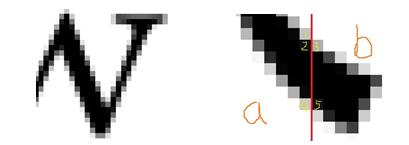（a）                                                                  （b）
图1：点阵字体的锯齿现象
由字体放大图1(a)可以看出笔画周边的锯齿，每一个锯齿方块就是一个像素，范围大小在255以下。切割后的图像，这些锯齿也会分开。但是，虽然像素分开了，由于汉字字体或者英文字体大部分是连体的，所以这些碎片的图像灰度信息会有一定的相关性或者说是连续性。
如图1(b)，假如图片像素按照红线所示切开分为a，b两块。可以看到红线左右两边的像素块大都是相连的，最理想的情况就是如同像素块2，3或者4，5在同一位置直接对应，这种情况就可以认为这两个像素块匹配，匹配数num加1。但是，也有可能出现像素块1这样的情况，与之对应的位置没有像素块，但是下方或者上方有像素块，由于这些像素的连续性，所以也认为像素1得到匹配，num=num+1。
读取所有图片，由于纸张有页边距，若某一张碎片为完整纸片上的最左一张，则其图像左侧必定全为白色，即灰度值的前几列为255，通过这种方法，能很快找到第一张碎片，将其放入集合中，记为。
找到第一张碎片后，用第一张碎片的右边缘去和中所有碎片的左边缘进行匹配。由于白色点太多，匹配成功数会很大，影响结果。所以，我们只选取非白色点进行匹配，匹配过程中，非白色点的总数记为sumofb，每选取边缘非白色点匹配一次，sumofb=sumofb+1。
匹配率的计算方法为：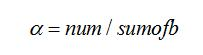（1）
求得第一张碎片的右边缘和集合中每张碎片左边缘的匹配率后，将所得的匹配率对比，选取匹配率最高的那张碎片，将其放入集合中，记为（i为碎片的排列顺序），将右边缘像素去和中剩余碎片的左边缘像素进行匹配，重复此类操作，直到中没有碎片，最终得到排序结果即为碎片的拼接方案。计算所得集合中与的最大匹配率如表1所示。

表1：附件一中中文字符碎片拼接顺序及匹配情况：

当前碎片编号  8  14  12  15  13  10  2  16  1  最佳匹配碎片编号  14  12  15  13  10  2  16  1  4  最大匹配率  0.83  0.96  0.82  0.92  0．97  0.92  0．97  0．94  0.83  当前碎片编号  4  5  9  13  18  11  7  17  0  最佳匹配碎片编号  5  9  13  18  11  7  17  0  6  最大匹配率  0.85  0.87  0.97  0.92  0.74  0.89  0.98  0.91  0.99

表2：附件一中英文字符碎片拼接顺序及匹配情况：

当前碎片编号  3  6  2  7  15  18  11  0  5  最佳匹配碎片编号  6  2  7  15  18  11  0  5  1  最大匹配率  0.93  0.91  0.95  0.92  0.89  0.90  0.76  0.96  0.88  当前碎片编号  1  9  13  10  8  12  14  13  16  最佳匹配碎片编号  9  13  10  8  12  14  13  16  4  最大匹配率  0.99  0.87  0.87  0.94  093  0.92  0.96  0.84  0.82

5.1.2  基于向量相关性的碎片拼接
提取碎片边缘向量，选出第一张图片后，计算右边缘向量与集合中的每一张碎片左边缘向量的相关系数：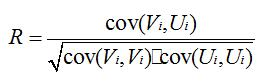（2）
将所得的对比，选出相关系数最最大的，放入集合中。再用选出的碎片右边缘向量与中剩余碎片匹配，重复此操作，直到中没有碎片，最终得到排序结果即为碎片的拼接方案。计算求得集合中与的相关系数为：
表3：附件二中中文字符碎片拼接顺序及匹配情况：

当前碎片编号  8  14  12  15  13  10  2  16  1  最相关碎片编号  14  12  15  13  10  2  16  1  4  最大相关系数  0.80  0.85  0.88  0.91  0．84  0.89  0．94  0．88  0.86  当前碎片编号  4  5  9  13  18  11  7  17  0  最相关碎片编号  5  9  13  18  11  7  17  0  6  最大相关系数  0.88  0.88  0.88  0.87  0.86  0.86  0.84  0.88  0.90

5．2  问题二的求解
问题二中所给附件三、四都是小碎片，宽度为72像素，与第一问中的一致，但是高度只有180像素，为第一问碎片的1/11，所以左右边缘的灰度信息较少，容易造成误判。
5.2.1 模型1 建立全局搜索的碎片拼接模型
由问题一的求解可以看出，利用向量相关模型或者文字连续性模型可以不必区分碎片的文字语言。所以对于问题二，可以建立全局搜索模型，同时利用第一问中的向量相关分析来进行碎片拼接。全局搜索模型算法如下：
Step 1：根据页边距找出11张处于纸片第一列的碎纸片，记为集合。取其中一张记为，放入；
Step 2：我们从存放于U的所有纸片中任取一张碎纸片与进行向量匹配，记录下匹配的相关系数，取相关系数最大的那张图片作为的下一张纸片，记为,以此法依次匹配得到后面的18张图片（最后一张同样存在页边距）；对于得到的这样一条横串，我们记为
Step 3：从上面的集合中再任取一张图片记为，重复step2；
Step 4：当，我们根据问题1中的方法对进行拼接，即可得到整张纸片原图。
5.2.2模型2 基于向量投影分类的碎片拼接模型
但是由于附件三和四中都给出了209张碎片，如果进行全局搜索的话，时间复杂度，计算量十分庞大。所以为了减少计算时间，我们首先根据碎片中行距的位置对碎片进行预分类，将同一行的碎片分在一类，再对同一类中的碎片利用第一文的方法进行排序。
由于中英文文字在像素图片中的显示形式不一样，所以我们通过这两种文字显示的不同特征来用两种投影方式来进行分类排序。
1、 中文字的碎片拼接
（1）中文字碎片向量投影
针对中文字像素图片，可以利用中文字是方块字的特点首先建立向量投影匹配分组模型。附件3中所给11×19共209张图片，可以拼成完整的一篇文章，那么同一行的文字被切开后，字体仍然在相对的同一高度上。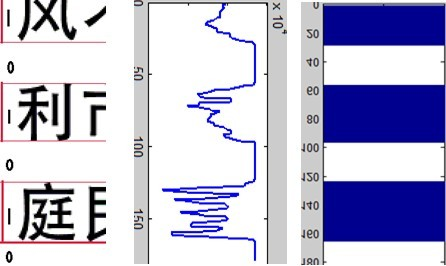图2 碎片投影
（2）字符的填补
投影碎片的灰度信息，由于中文字中有上下结构的字，如，附件三中的“010.bmp”碎片中的“翁”字，中间有一小段的投影出现缺失。这时，由于投影向量中全白色段的长度很小（甚至可能为1个像素点），并且上下两字段的长度加起来接近一个中文字符块的高度时，我们将此段的投影向量填充为1，似得其投影矩阵具有连续性。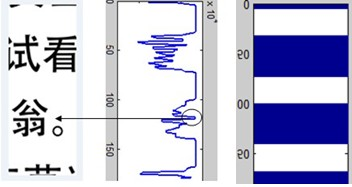图3 字符缺损补充
（3） 字块的填补
利用掩码补充模型补充缺行碎片。在投影过程中，会遇到缺行的图片，这种缺行的图片大致分为三种：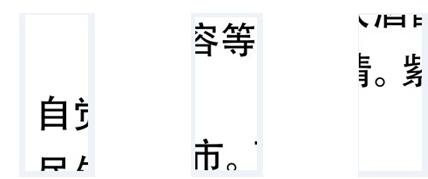图4 掩码补充时的特殊情况
如图4所示，有上部缺行，中部缺行和底部缺行。如果出现这样的情况，因为若全为白色的像素行过多，就有可能匹配不到分组。所以我们建立掩码补充模型，补充缺失的文字像素投影行，将缺失的文字像素投影段都补充为1，每一列的投影矩阵都叫做“投影编码”。
首先，我们将缺失投影行的图片搜寻出来，由于缺失一个字段，那么这些图片的向量投影值为1的连续段就会小于3，只有两段甚至是一段。利用这个方法，我们成功找出了缺失字段的图片，分别是第5 9 15 17 22 2628 33 41 61 67 71 72 75 86 90 94 102 107 109 110 111 114 115 118 120 124 126140 141 146 147 151 153 154 155 156 158 166 167 174 182 185 186 188 195 197 198205 206 208共51张（此数列的值比实际图片编号大一，如，第五张对应附件三中的004号图片）图片。
然后，我们将这些缺损字段的图片投影数据进行整合，计算每一段全白色段和非全白色段的段长（例如投影编码为00000011111100011，就记为6，6，3，2）并存入段长矩阵中，为了以后处理方便，我们都以全白色段的段长值开头，若起始为非纯白色段，则在此段长前加一0值，作为开头的全白色段长。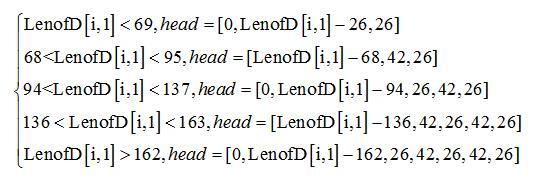（3）
对于中部缺行的图片我们也采取类似的方案进行处理。对于图片中间部分的全白色段段长LenofD[i,mid],因为中部缺损肯定的是缺[非全白色段，全白色段，非全白色段]或者是[非全白色段，全白色段，非全白色段，全白色段，非全白色段]，只有两种情况，所以当LenofD[i,mid]大于（2×Dz+Hz）时：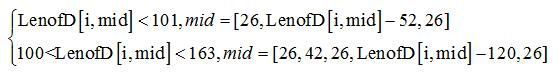（4）
用mid矩阵取代LenofD[i,mid]。
最后一种底部缺行的处理方案也与上部缺行的类似。如果图片底部的LenofD[i,bom]大于间距Dz，就将LenofD[i,bom]分解成小块段长：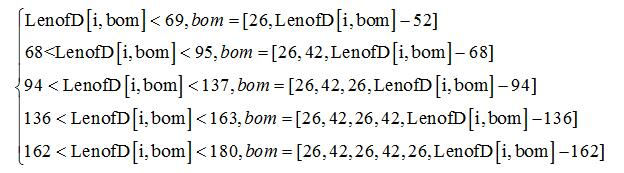（5）
用bom矩阵取代LenofD[i,bom]。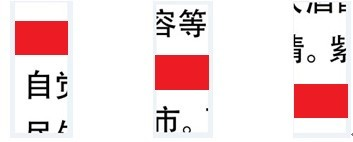图5 掩码遮盖
（4）用FCM聚类方法将碎片分类
表4：FCM预分类结果
C1  6     11    30    38    45    49    56    60    65    76    93    99    105  112  172  173  181  202  207  C2  7     20    21    37    53    62    64    68    70    73    79    80    97    100  117  132  163  164  178  C3  3     12    23    29    50    55    58    66    92    96    119  130  142  144  179  187  189  191  193  C4  9     10    25    26    36    39    47    75    82    89    104  106  123  131  149  162  168  190  194  C5  1     8     33    46    54    57    69    71    94    127  138  139  154  159  167  175  176  197  209  C6  17    22    67    107  110  111  140  146  151  158  174  182  185  188  198  205  C7  35    43    44    48    59    78    85    91    95    98    113  122  125  128  137  145  150  165  184  C8  4     13    15    32    40    52    74    83    108  116  129  135  136  160  161  170  177  200  204  C9  14    16    18    28    34    72    81    84    86    133  134  153  157  166  171  183  199  201  203  206  C10  2     19    24    27    31    42    51    63    77    87    88    101  121  143  148  169  180  192  196  C11  5  41  61     90    102  103  109  114  115  118  120  124  126  141  147  152  155  156  186  195 208
分类后C6中只有16张图片（少3张），C9中有20张（多1张），C11中有21张（多2张）。
（5）将分类好的碎片逐类横向拼接
分类完毕之后，继续利用向量相关模型进行横向拼接，拼接好同一类的一行之后，观察是否有拼接出错碎片。此时，由于程序对有些碎片无法正确匹配，必须进行人工干预，根据语义和偏旁部首进行手工拼接。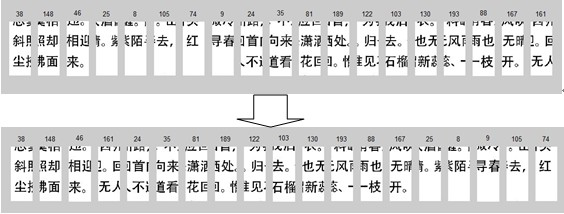图6 人工干预前后比较
如图6，第46张与第25张碎片匹配出错，因为“迎”和“晴”都切在了竖线上，所以相关系数会很大，这是第一类典型的出错原因：边缘字形相近；第74张与第9张匹配出错，因为第74张碎片的右端边缘没有任何中文字符，造成正确无法计算相关系数，这是第二类出错原因：碎片边缘没有任何字符灰度信息。
人工干预时，根据中文字的特征，以及上下文的语义，我们进行手动调整错误的图片的位置，将此行拼成正确图片。
当每一个分类都成功拼接出正确图片行后，将这11个分类用在纵向进行拼接。纵向拼接成功后，在用向量相关模型进行横向拼接，最终得到正确结果：
表5：附件三的拼接结果

49  54  65  143  186  2  57  192  178  118  190  95  11  22  129  28  91  188  141  61  19  78  67  69  99  162  96  131  79  63  116  163  72  6  177  20  52  36  168  100  76  62  142  30  41  23  147  191  50  179  120  86  195  26  1  87  18  38  148  46  161  24  35  81  189  122  103  130  193  88  167  25  8  9  105  74  71  156  83  132  200  17  80  33  202  198  15  133  170  205  85  152  165  27  60  14  128  3  159  82  199  135  12  73  160  203  169  134  39  31  51  107  115  176  94  34  84  183  90  47  121  42  124  144  77  112  149  97  136  164  127  58  43  125  13  182  109  197  16  184  110  187  66  106  150  21  173  157  181  204  139  145  29  64  111  201  5  92  180  48  37  75  55  44  206  10  104  98  172  171  59  7  208  138  158  126  68  175  45  174  0  137  53  56  93  153  70  166  32  196  89  146  102  154  114  40  151  207  155  140  185  108  117  4  101  113  194  119  123

2、英文字的碎片拼接
（1）英文字碎片向量投影
对于英文字母，我们可以利用英文字母“四线三格”的书写格式，如图7所示：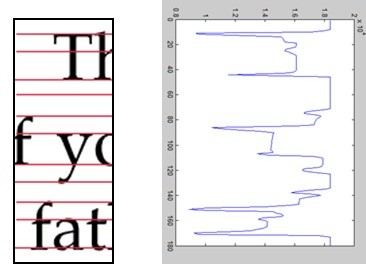图7 英文字符“四线三格”表示
这种书写格式我们也可以通过碎片灰度矩阵的每行灰度值的和表示成折线图，从中图7
观察出来。每当那一行的值变化比较剧烈时，那一行一般情况下总是四线中的某一线。
通过多次分析得出相邻两条直线之间的距离，大致分别为(12,20,15,25),之后制作模板，把每一个碎片与模板比较。
通过寻找每一个碎片起始直线位置，与模板对应寻找可以匹配的个数，通过循环找出与模板匹配率最高的模式。根据匹配的模式，从起始位置对碎片根据此模式的间距向上向下添加直线，完成直线填补工作。
之后，通过模糊C均值(FCM)聚类方法进行分类，根据补齐直线投影矩阵分出11类，由于分类方法的模糊性特征，造成分出的类别误差相对较大（如下），在之后运用相关性函数匹配时造成了很大的错误，在人工干预时大大增加了工作量。
表6：英文碎片分类结果
C1  7     15    18    24    48    55    61    62    63    69    71    85    91    92    97    100  101  102  104  123 138  147  149  157  173  175  186  196  197  199  209  C2  27    29    34    143  163  170  C3  3     5     12    22    33    40    65    66    68    76    105  107  113  120  137  148  150  155  180  181 185  190  191  192  193  198  205  C4  8     50    119  134  169  C5  10    11    17    20    45    57    58    67    72    83    84    93    94    106  122  127  135  142  146  153 158  172  177  183  184  195  203  206  C6  19    23    28    35    36    43    56    75    89    111  115  152  156  166  168  C7  16    21    37    42    44    46    74    77    78    80    103  109  117  124  136  141  144  162  174  200 208  C8  1     2     49    51    53    54    64    73    82    86    88    90    98    116  121  125  126  129  130  132 139  140  154  160  161  176  178  188  194  201    C9  4     6     14    25    31    38    41    47    52    59    108  112  114  118  128  131  133  145  151  159 164  179  187  189  202  207  C10  13    32    39    60    70    79    95    96    99  165  167  204  C11  9     26    30    81    87    110  171  182
尽管直线修补后误差依然不小，相比于直接模糊C均值(FCM)聚类方法分类，大大提高了成功率，所以可以根据英文字书写格式的这种特征来进行向量投影。
表7：附件四的拼接顺序

191  75  11  154  190  184  2  104  180  64  106  4  149  32  204  65  39  67  147  201  148  170  196  198  94  113  164  78  103  91  80  101  26  100  6  17  28  146  86  51  107  29  40  158  186  98  24  117  150  5  59  58  92  30  37  46  127  19  194  93  141  88  121  126  105  155  114  176  182  151  22  57  202  71  165  82  159  139  1  129  63  138  153  53  38  123  120  175  85  50  160  187  97  203  31  20  41  108  116  136  73  36  207  135  15  76  43  199  45  173  79  116  179  143  208  21  7  49  61  119  33  142  168  62  169  54  192  133  118  189  162  197  112  70  84  60  14  68  174  137  195  8  47  172  156  96  23  99  122  90  185  109  132  181  95  69  167  163  166  188  111  144  206  3  130  34  13  110  25  27  178  171  42  66  205  10  157  74  145  83  134  55  18  56  35  16  9  183  152  44  81  77  128  200  131  52  125  140  193  87  89  48  72  12  177  124  0  102  115

根据不同的文字特征，对中英文碎片采取了不同的数学模型，汉字属于方块字，有明显的笔画连续性，字宽、字高，通过对方块字字块的判断可以比较好的判断匹配率；英文有明显的四线三格模式，通过直线寻找可以很好地把英文特征识别，提高匹配率。
5.3问题三的求解
5.3.1 基于组合匹配模型的双面碎片拼接
针对第三问附件中碎片的正反面，若某一碎片正面与另一碎片正面能够匹配，那么这两张图片的反面就是匹配的。利用这一特性，我们可以建立组合匹配模型。
将碎片图像信息转化为灰度矩阵，将每张图片的a，b面所对应的灰度矩阵上下拼接，每张碎片的拼接方案有两种（灰度矩阵a放在左右镜像处理后的b上或灰度矩阵b放在左右镜像处理后的a上），效果如图8所示：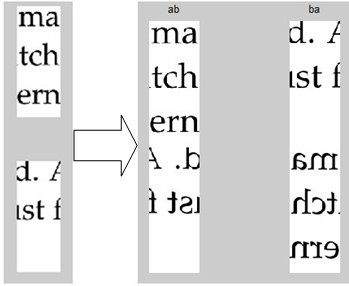图8 拼接方案
通过拼接大大增加碎片的信息量，在接下来的模式匹配寻找，行列拼接时提高了成功率。
拼接之后，延用第二问的全局搜索模型。首先，搜索碎片的行首、行尾放到两个数组中，并把行首、行尾为之标记；接着，全文搜索，运用相关系数函数匹配，寻找出与之匹配率最高的碎片，放进一个集合中。
由于灰度矩阵的相似性，匹配时出现错误，形成一段一段拼接好的碎片图像，观察每一行用肉眼可以看出的错误的位置，并进行调整，最后，把每一行的碎片拼接完成。每一行拼接好之后，假设a面为正面，b面为反面，把b面的灰度矩阵连接到a面后面，形成Z（a，b）灰度矩阵；如果b为正面，a为反面，形成Z（b，a）灰度矩阵。
把每一行的灰度矩阵，之后把灰度矩阵进行转置，运用第一问的碎片相关性方法对灰度矩阵进行匹配，最后得出正确结果：
表8：附件五碎片正面拼接顺序

136a  047b  020b  164a  081a  189a  029b  018a  108b  066b  110b  174a  183a  150b  155b  140b  125b  111a  078a  005b  152b  147b  060a  059b  014b  079b  144b  120a  022b  124a  192b  025a  044b  178b  076a  036b  010a  089b  143a  200a  086a  187a  131a  056a  138b  045b  137a  061a  094a  098b  121b  038b  030b  042a  084a  153b  186a  083b  039a  097b  175b  072a  093b  132a  087b  198a  181a  034b  156b  206a  173a  194a  169a  161b  011a  199a  090b  203a  162a  002b  139a  070a  041b  170a  151a  001a  166a  115a  065a  191b  037a  180b  149a  107b  088a  013b  024b  057b  142b  208b  064a  102a  017a  012b  028a  154a  179b  158b  058b  207b  116a  179a  184a  114b  035b  159b  073a  193a  163b  130b  021a  202b  053a  077a  016a  019a  092a  190a  050b  201b  031b  171a  146b  172b  122b  182a  040b  127b  188b  068a  008a  117a  167b  075a  063a  067b  046b  168b  157b  128b  195b  165a  105b  204a  141b  135a  027b  080a  000a  185b  176b  126a  074a  032b  069b  004b  077b  148a  085a  007a  03a  009a  145b  082a  205b  015a  101b  118a  129a  062b  052b  071a  033a  119b  160a  095b  051a  048b  133b  023a  054a  196a  112b  103b  055a  100a  106a  091b  049a  026a  113b  134b  104b  006b  123b  109b  096a  043b  099b

表9：附件五碎片反面拼接顺序

078b  111b  125a  140a  155a  150a  183b  174b  110a  066a  108a  018b  029a  189b  081b  164b  020a  047a  136b  089a  010b  036a  076b  178a  044a  025b  192a  124b  022a  120b  144a  079a  014a  059a  060b  147a  152a  005a  186b  153a  084b  042b  030a  038a  121a  098a  094b  061b  137b  045a  138a  056b  131b  187b  086b  200b  143b  199b  011b  161a  169b  194b  173b  206b  156a  034a  181b  198b  087a  132b  093a  072b  175a  097a  039b  083a  088b  107a  149b  180a  037b  191a  065b  115b  166b  001b  151b  170b  041a  070b  139b  002a  162b  203b  090a  114a  184b  179b  116b  207a  058a  158a  179a  154b  028b  012a  017b  102b  064b  208a  142a  057a  024a  013a  146a  171b  031a  201a  050a  190b  092b  019b  016b  077b  053b  202a  021b  130a  163a  193b  073b  159a  035a  165b  195a  128a  157a  168a  046a  067a  063b  075b  167a  117b  008b  068b  188a  127a  040a  182b  122a  172a  03b  007b  085b  148b  077a  004a  069a  032a  074b  126b  176a  185a  000b  080b  027a  135b  141a  204b  105a  023b  133a  048a  051b  095a  160b  119a  033b  071b  052a  062a  129b  118b  101a  015b  205a  082b  145a  009b  099a  043a  096b  109a  123a  006a  104a  134a  113a  026b  049b  091a  106b  100b  055b  103a  112a  196b  054b

六．模型结果的分析与检验
6.1 连续性模型的实验分析检验
利用文字连续性模型，最终成功将附件一和附件二的长条型中英文字体碎片很好的拼接起来了，拼接后的文章语意通顺，说明结果正确。由于问题一中的纵向切割，使得形成的边缘处除了第一张和最后一张外，其余碎片没有完全空白的情况，这给我们寻找第一张碎片带来了很大的便利。这种先找出第一张碎片，再继续拼接后续碎片的方法。可以大大简化边缘匹配的过程。
经过程序实验论证，针对问题一的中英文碎片拼接，拼接后的正确率为100%。
这种连续性模型可以很好的拼接出边缘所含信息较多的碎片。但如果对于边缘只含有少量文字或者与其他碎片含有相似字形较多的碎片，就会产生误差。
所以这种模型适用于边缘信息较多的碎片拼接。
6.2  全局搜索模型以及向量投影分组模型的分析检验
问题二中所给附件三、四都是小碎片，宽度为72像素，与第一问中的一致，但是高度只有180像素，为第一问碎片的1/11，所以左右边缘的像素信息也只为附件一和二的1/11,如果实用文全局搜索模型利用向量相关性的方法来拼接碎片的时间复杂度。
而如果使用向量投影分组模型，把每一行有黑点的情况投影到一个数组中，组成以一个180*209的数组，之后运用聚类分析方法分出11类，分类时由于空白处造成分类误差，运用相关系数函数匹配判断时出现匹配错误后，运用人工干预，分为11类。然后进行横向匹配，此时的时间复杂度只为。此模型能节约大量运算时间并且能较好得适应边缘特征并不明显的时候，但由于分类的误差，造成最后匹配时出现了错误，需要人工干预，增加了工作量。
6.3  组合匹配模型的分析检验
通过图片的组合拼接，把碎片的信息量进行扩充，增大信息量，图片特征，在下面的对每一行的分类，每一列的匹配拼接提高了成功率。并通过反面对正面的检测分析，可以更好的避免误差，减少人工干预的次数，减少了工作量。但由于使用的是全局搜索模型，在时间复杂度，耗时较多。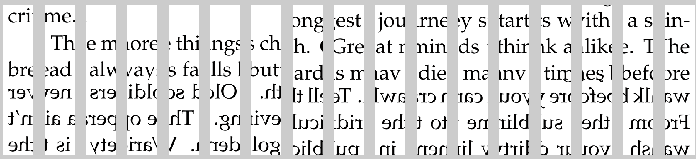图9附件五碎片横向拼接
根据碎片拼接图片可以看出，有碎片拼接错误，主要缘由是匹配函数的选取，相关性的匹配，近似灰度矩阵就会造成干扰。使得最后匹配的数据出现了错误，造成一段一段的碎片，给人工干预时造成了一定的麻烦。通过反面的拼接去检验正面拼接的正确性。
七．模型的评价与推广
7．1 模型的评价
（1）问题一中的连续性模型对于拼接边缘信息量较大的碎片有较高的效率以及很高的准确率，但是当单独用该模型解决例如附件三、四的小碎片时，虽然效率很高，但是准确率较低。比如，如果有两张或者多张图片边缘切割都为白色时，可能就会出现无法匹配的情况，这时就需要人工干预；向量相关模型相对于文字连续性模型，具有更高的准确度。
（2）问题二中的全局搜索模型不仅耗时多，而且由于给的信息很少，在这么多图片中匹配，会造成准确率很低的情况；向量投影分组模型，投影图片灰度向量到投影矩阵中，利用FCM聚类分析方法让打乱的横切及纵切的图片得以分类，从而分步实现了碎片的排序，简化了拼接过程，大大减少了循环匹配的次数；
（3）问题三中组合匹配模型将附件五中的a，b两图片上下拼接，然后用拼接后的图片进行分类，这样不仅提高了效率，还一定程度上增加了碎片所提供的信息。
7. 2 模型的推广
这三种模型针对不同的情况，利用多种模型和匹配方法，能够较好的解决题目中的三个问题。
但是，我们仍然可以考虑其他特征，结合连续性模型或者向量相关模型综合考虑，比如字宽特征，计算两边的碎片边缘被切开字的缺损字宽，两边相加，当和值与标准字宽相差较大时，就可以排除不利因素。
现在已经有很多模式识别的算法和软件，我们可以利用文字识别算法来计算拼接后的两片碎片上的文字上的准确率，当准确率较高时，则认为匹配成功。利用已有的技术，可以大大效率有准确率
八．参考文献
 章毓晋. 图像处理（第三版）. 清华大学出版社，2012.2
 边肇祺，张学工. 模式识别（第二版）.清华大学出版社 2000.1
 冯宇平,戴明,孙立悦，张威. 图像自动拼接融合的优化设计.光学精密工程.2012,18（2）
 李仁发，杨高波. 特征提取与图像处理（第二版）.电子工业出版社 2010.10
 陈丽莉，刘贵喜.一种有效的序列图像自动拼接方法. 光电子激光. 2011,22（7）

九．附录
附录一：程序代码
% 第一问代码 文件名：Qusetion1.m
files=dir('C:\Users\ZYF\Desktop\新建文件夹\B\附件1\*.bmp');    %批量载入图像
for n=1:numel(files)
end
[h,z]=size(image{1,1});
for k=1:n
byz(:,k)=image{1,k}(:,1);      %将第k张图片的最左边一列放入矩阵byz的第k列
byy(:,k)=image{1,k}(:,z);      %将第k张图片的最右边一列放入矩阵byy的第k列
end
%利用页边距寻找第一张图片
for i=1:n
sum=0;
for j=1:h
if byz(j,i)==255
sum=sum+1;
else
break;
end
end
if sum==h
f=i;
end
end
paixu=zeros(1,n);
index=1;
paixu(index)=f;

%依据连续性模型需找匹配点
for i=2:n
max=0;
for j=1:n
sumofbp=0;
num=0;
for k=2:h-1
ifbyy(k,paixu(index))~=255
ifbyz(k,j)<255||byz(k-1,j)<255||byz(k+1,j)<255
num=num+1;
else
num=num;
end;
end
end
if num>max
max=num;
signal=j;
end
end
index=index+1;
paixu(index)=signal;               %存放排好序的图片序号
end
temp=image{paixu(1)};
for i=2:n
temp=[tempimage{paixu(i)}];       %将排序好的图片存放与temp
end
imshow(temp)                           %完整显示整张纸片

%第二问中文拼接中投影代码   文件名reflect.m
% 碎片预处理，图像投影，存放投影数组
files=dir('C:\Users\ZYF\Desktop\新建文件夹\B\附件3\*.bmp');%载入图像
figure;
A=zeros(1,180);
for n=1:numel(files)
end
[x,y]=size(image{1});
flag1=zeros(x,n);
for i=1:n
for j=1:x
sum=0;
for k=1:y
ifimage{i}(j,k)<255
break;
else
sum=sum+1;
end
end
if sum==y
flag1(j,i)=0;
else
flag1(j,i)=1;
end
end
end

%如果采用掩码模型，则运行代码yanma.m（包含3个函数top.m,mid.m,back.m）
%附件三掩码补充代码   文件名   yanma.m
files4=dir('C:\Users\asus\Desktop\2013B3\附件3\*.bmp');%载入图像
for n4=1:numel(files4)
end
fid1=fopen('C:\Users\asus\Desktop\数模数据.txt','wt');
fid2=fopen('C:\Users\asus\Desktop\需补充.txt','wt');
[x,y]=size(image4{1});
flag=zeros(x,n4);
%向量投影
for i=1:n4
for j=1:x
sum=0;
for k=1:y
ifimage4{i}(j,k)<255
break;
else
sum=sum+1;
end
end
if sum==y
flag(j,i)=0;
else
flag(j,i)=1;
end
end
end

sumofb=0;
for i=1:209
sumofb=0;
for j=1:179
ifflag(j,i)==1&&flag(j+1,i)==0
sumofb=sumofb+1;
else
sumofb=sumofb;
end
end
if flag(180,i)==1
sumofb=sumofb+1;
end
numofb(i)=sumofb;
end
for i=1:209
if numofb(i)<3
fprintf(fid2,'%g ',i);
end
end
flagnew=flag;
A=[5 9 15 17 22 26 28 33 41 61 67 71 72 75 86 90 94 102 107 109 110111 114 115 118 120 124 126 140 141 146 147 151 153 154 155 156 158 166 167 174182 185 186 188 195 197 198 205 206 208];
[ax,ay]=size(A);
shul=zeros(ay,6);
for i=1:ay%统计投影白色0和黑色1的数量
sumofd=0;
index=1;
if flag(1,A(i))==1%若第一个为黑色，则白色个数为0
shul(i,index)=0;
index=index+1;
end
for j=1:179
ifflag(j,A(i))==flag(j+1,A(i))
sumofd=sumofd+1;
else
sumofd=sumofd+1;
shul(i,index)=sumofd;
index=index+1;
sumofd=0;
end
end
shul(i,index)=sumofd+1;
end
for i=1:ay
if shul(i,1)>26
topout=top(shul(i,1));
hebin(i,:)=[topoutshul(i,2:6)];
end
end
for i=1:ay
for j=3
if shul(i,j)>31
midout=mid(shul(i,j));
hebin(i,:)=[shul(i,1:2) midout shul(i,4:6)];
end
end
end
for i=1:ay
for j=5
if shul(i,j)>26
backout=back(shul(i,j));
hebin(i,:)=[shul(i,1:4) backout];
end
end
end
for i=1:ay
if hebin(i,1)~=0
temp=hebin(i,1);
else
temp=1;
end
for j=2:2:6
flagnew(temp:(temp+hebin(i,j)),A(i))=1;
temp=temp+hebin(i,j)+hebin(i,j+1);
end
end

%top函数代码   文件名top.m
%top函数
function [ topout ] = top( in)
if in<69
topout=[0 in-26 26];
elseif 68<in<95
topout=[in-68 42 26];
elseif 94<in<137
topout=[0 in-94 26 42 26];
elseif 136<in<163
topout=[in-136 42 26 4226];
else
topout=[0 in-162 26 42 2642 26];
end
end

%mid函数   文件名   mid.m
%mid函数
function [ midout ] = mid( in )
if in<101
midout=[26 in-52 26];
elseif 100<in<163
midout=[26 42 26 in-12026];
end
end

%back函数   文件名 back.m
%back函数
function [ backout ] = back( in )
if in<69
backout=[26 in-26 0 0];
elseif 68<in<95
backout=[26 42 in-68 0];
elseif 94<in<137
backout=[26 42 26 in-94];
end
end

%第二问C均值分类代码  文件名Classify.m
%模糊C均值（fcm）分类
clear;
close all;
files=dir('C:\Users\ZYF\Desktop\新建文件夹\B\附件3\*.bmp');%载入图像
figure;
for n=1:numel(files)
end
data = flag1';
[center,U,obj_fcn] = fcm(data, 11);
maxU = max(U);
%index(i)中存放第i类分组的图片
index(1).t = find(U(1,:) == maxU);
index(2).t = find(U(2, :) == maxU);
index(3).t = find(U(3,:) == maxU);
index(4).t = find(U(4,:) == maxU);
index(5).t = find(U(5,:) == maxU);
index(6).t = find(U(6,:) == maxU);
index(7).t = find(U(7,:) == maxU);
index(8).t = find(U(8,:) == maxU);
index(9).t = find(U(9,:) == maxU);
index(10).t = find(U(10,:) == maxU);
index(11).t= find(U(11,:) == maxU);

%第二问中文拼接代码   文件名Question_Chinese.m
clear;
close all;
% 问题二汉字匹配拼接
class=12;
files=dir('C:\Users\ZYF\Desktop\新建文件夹\B\附件3\*.bmp');%载入图像

for n=1:numel(files)
end

for k=1:n
bz(:,k)=image{1,k}(:,1);                 %将第k张图片的最左边一列放入矩阵bz的第k列
br(:,k)=image{1,k}(:,72);               %将第k张图片的最右边一列放入矩阵br的第k列
end
ind=1;
for oo=1:class
tmp=[];
first=index(oo).t;                      %将index分组的第oo组存放入first矩阵
cd=length(first);
f=zeros(1,cd);                         %标记图片是否已被使用，初始为0
kk=0;
for j=1:cd
sum=0;
for p=1:180
for q=1:5
ifimage{first(1,j)}(p,q)==255
sum=sum+1;
end
end
end
if sum==900
figure (oo);
subplot(1,cd,1)
imshow( image{first(j)});
kk=first(j);
f(1,1)=first(j);                 %已用图片
break;
end
end
jj=1;
if(kk==0)
figure (oo);
subplot(1,cd,1)
imshow( image{first(1)});
kk=first(1);
f(1,1)=first(1);                % 已用图片
end
tmp=[tmp kk];
sign=kk;
for z=1:cd
max=0;
for a=1:cd
sum1=0;
hh=0;
for ll=1:cd
if(first(a)==f(1,ll))
hh=1;
end
end
if(hh==0)
co=corrcoef(double(bz(:,first(a))),double(br(:,kk)));% 相关性函数判断
sum1=abs(co(1,2));
if(max<sum1)
max=sum1;
sign=first(a);
end
end
end

if(kk ~= sign)
tmp=[tmp sign];
subplot(1,cd,jj+1)
imshow(image{sign});
kk=sign;                          %现在图片
f(1,jj+1)=sign;
jj=jj+1;
else
for d=1:cd
for s=1:cd
gg=0;
if(first(d) ==f(1,s))
gg=1;
end
end
if(gg==0)
kk=first(d);
break;
end
end
cc(ind).a=tmp;
ind=ind+1;
tmp=[kk];
end
end
end

%第二问英文添加直线代码  文件名  Line.m
% 英文直线的修补
clear;
close all;
files=dir('.\附件4\*.bmp');%载入图像
for n=1:numel(files)
A(n).t=sum(image{n},2);
end

B=[12,20,15,25,12,20,15,25];%做模板
B1=[12,20,15,25,12,20,15,25,12,20,15,25];
B2=[20,15,25,12,20,15,25,12,20,15,25,12];
B3=[15,25,12,20,15,25,12,20,15,25,12,20];
B4=[25,12,20,15,25,12,20,15,25,12,20,15];
idx1=zeros(600,1);% 模板数组
idx1(1,1)=1;
mm=1;
for i=1:6
for j=1:4
mm=mm+B(j);
idx1(mm,1)=1;
end
end

%ind=A(10).t;
BZ=zeros(180,209);
for pp=1:209
ind=A(pp).t;
for i=2:179   % 拐点找出
if(ind(i-1)-ind(i)>10 &&ind(i+1)-ind(i)>10)
BZ(i,pp)=1;
end
end
if ind(1)<ind(2)
BZ(1,pp)=1;
end
if ind(180)<ind(179)
BZ(180,pp)=1;
end
po=find(BZ(:,pp)==1);
gg=1;
max=0;
for ii=1:5  % 与模板匹配
dd=0;
fh=0;
for z=po(1):180
if(BZ(z,pp)==1&&idx1(z+gg,1)==1||z+1<=180&&BZ(z+1,pp)==1&&idx1(z+gg,1)==1||(z-1)~=0&& BZ(z-1,pp)==1&&idx1(z+gg,1)==1)% 与模板匹配
dd=dd+1;
end
end
if(max<dd)
SG=ii;
max=dd;
kh=po(1);
end
gg=gg+B(ii);
end

if SG==1||SG==4
AB=kh;
j=1;
while AB-B1(5-j)>=1
BZ(AB-B1(5-j))=1;
AB=AB-B1(5-j);
j=mod(j+1,4);
end
j=1;
j=j+1;
end
end
if SG==2
AB=kh;
j=1;
while AB-B2(5-j)>=1
BZ(AB-B2(5-j))=1;
AB=AB-B2(5-j);
j=mod(j+1,4);
end
j=1;
j=j+1;
end
end
if SG==3
AB=kh;
j=1;
while AB-B3(5-j)>=1
BZ(AB-B3(5-j))=1;
AB=AB-B3(5-j);
j=mod(j+1,4);
end
j=1;
j=j+1;
end
end
if SG == 4
AB=kh;
j=1;
while AB-B4(5-j)>=1
BZ(AB-B4(5-j))=1;
AB=AB-B4(5-j);
j=mod(j+1,4);
end
j=1;
j=j+1;
end
end
end

%第二问英文拼接主代码  文件名  Question.m
clear;
close all;
class=12;
files=dir('C:\Users\ZYF\Desktop\新建文件夹\B\附件4\*.bmp');%载入图像
for n=1:numel(files)
end
for k=1:n
bz(:,k)=image{1,k}(:,1); %首行
br(:,k)=image{1,k}(:,72);%末行
end
ind=1; % 类别计数器
for oo=1:class
dx=0;   %  倒序寻找
tmp=[];
first=index2(oo).t;
cd=length(first);
f=zeros(1,length(first));  %标记
kk=0;
while (sum(f)<length(first))
if length(tmp)==0            % 找行首块
for j=1:length(first)
if f(j)==0
im=image{ first(j)};
sumim=sum(im,1);
ifsum(sumim(1:10))==255*180*10  % 找到行首
figure (oo);
subplot(1,length(first),1)
imshow( image{first(j)});
kk=first(j);
f(j)=1;% 标记已用图片
break;
end
end
end
% 找块尾
for j=1:length(first)
if f(j)==0
im=image{ first(j)};
sumim=sum(im,1);
ifsum(sumim(70:72))==255*180*3  % 找到行尾
figure (oo);
subplot(1,length(first),1)
imshow( image{ first(j)});
kk=first(j);
f(j)=1;% 标记已用图片
dx=1;
break;
end
end
end
if(kk==0)  % dou找不到，用第可用一个图片
figure (oo);
subplot(1,length(first),1);
imshow( image{ first(1)});
pos=find(f==0);
kk=first(pos(1));
f(pos(1))=1; % 标记已用图片
end
tmp=[tmp kk];
end
maxsum=0; % 找最接近的
sign=kk;
for z=1:length(first)
if f(z)==0   % 没有用过
sum1=0;
for p=1:180
if dx==1
cha=abs(bz(p,kk)-br(p,first(z)));
if ( cha ==0 &&bz(p,kk)~= 255 && br(p,first(z))~=255)
sum1=sum1+1;
end
else
cha=abs(br(p,kk)-bz(p,first(z)));
if ( cha <20&& br(p,kk)~= 255 && bz(p,first(z))~=255)
sum1=sum1+1;
end
end
end
if(maxsum<sum1)
maxsum=sum1;
fpos=z;
sign=first(z);
end
end
end
if maxsum<1
sign=kk;
end
if(kk~=sign)
%找到了
if dx==1
tmp=[sign tmp ];
else
tmp=[tmp sign];
end
imshow(image{sign});
kk=sign;%现在图片
f(fpos)=1;
else            %没找到
cc(ind).a=tmp;ind=ind+1;
kk=0;tmp=[];dx=0;
end
end
end
for i=1:length(cc)
figure(20);clf;
for j=1:length(cc(i).a)
subplot(1,length(cc(i).a),j);
imshow(image{cc(i).a(j)});
end
pause;
end

%第三问英文拼接主代码   文件名  Question3_English.m
clc,clear;
% 碎片匹配连接
for n=1:209
fnamea=[num2str(n-1,'%03d') 'a.bmp'];
fnameb=[num2str(n-1,'%03d') 'b.bmp'];
image5a{2*n-1}=[image5{n}.a;fliplr(image5{n}.b)];%碎片a、b组合连接
image5a{2*n} =[image5{n}.b;fliplr(image5{n}.a)];
end
figure(1);clf;
subplot(2,1,1);imshow(image5{1}.a);
subplot(2,1,2);imshow(image5{1}.b);
figure(2);clf;
subplot(1,2,1);imshow(image5a{1});title('ab');% 显示组合效果
subplot(1,2,2);imshow(image5a{2});title('ba');
save image5.mat;
n=209;
for k=1:2*n
bz(:,k)=image5a{1,k}(:,1);
br(:,k)=image5a{1,k}(:,72);
end
first=zeros(1,22);
last=zeros(1,22);
flag=zeros(2*n,1);
for i=1:2*n
im=image5a{i};
sim=sum(im,1);
if sum(sim(1:5))==360*255*5
flag(i)=1;  % 标记行首
end
if sum(sim(72-4:72))==360*255*5
flag(i)=2; % 标记行尾
end
end
first=find(flag==1);
last=find(flag==2);
ind=1;
flg=zeros(2*n,1); % 判断是否被用过
for hh=1:length(first)
tmp=[];
k=first(hh);
tmp=[tmp k];
flg(k)=1;
ry=1;% 另起一行的标志
while ry == 1
maxsum=0;
for i=1:2*n
sum1=0;
if( (flag(i)~= 1) && (flg(i)==0) )
co=corrcoef(double(bz(:,i)),double(br(:,k)));
sum1=abs(co(1,2));
if(maxsum<sum1)
maxsum=sum1;
sign=i;
end
end
end
if(k ~= sign) % 找到了
tmp=[tmp sign]
k=sign; % 现在图片
flg(k)=1;
ry=1;
else
cc(ind).a=tmp; % 没找到
ind=ind+1;
ry=0;
end
end
end
save cc.mat cc
% for i=1:length(cc)
%    figure(i);clf;
%    for j=1:length(cc(i).a)
%        leibie(i,j)=cc(i).a(j);
%        subplot(1,length(cc(i).a),j);
%        imshow(image5a{cc(i).a(j)});
%    end
% end

%第三问英文拼接图片显示代码   文件名  Question3show.m
% 附件5碎片拼接后，每一行的显示
close all;
for i=1:length(cc)
figure(i);clf;
for j=1:length(cc(i).a)
leibie(i,j)=cc(i).a(j);
subplot(1,length(cc(i).a),j);
imshow(image5a{cc(i).a(j)});
end
end

附件二：实验结果
附件1结果：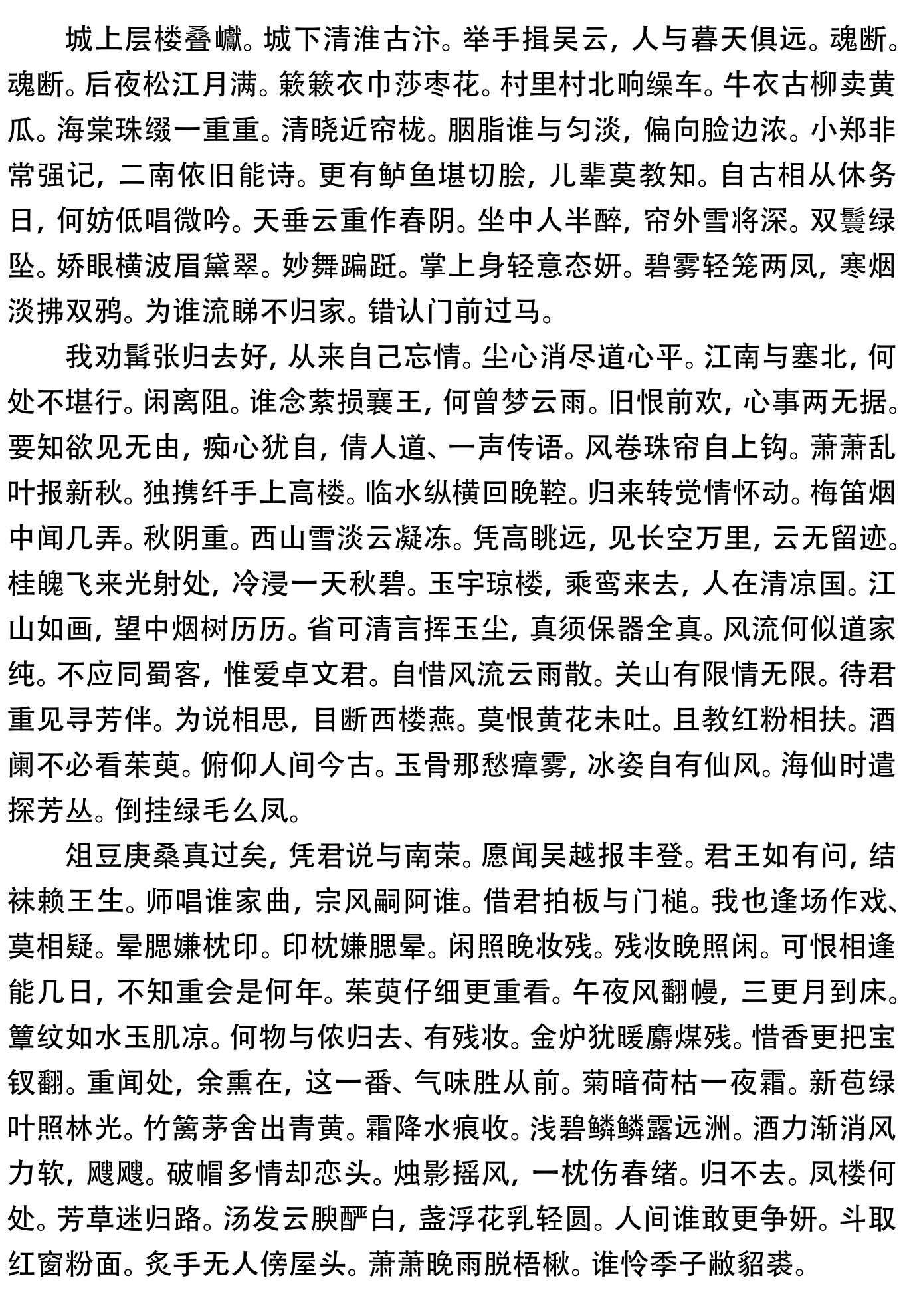附件2结果：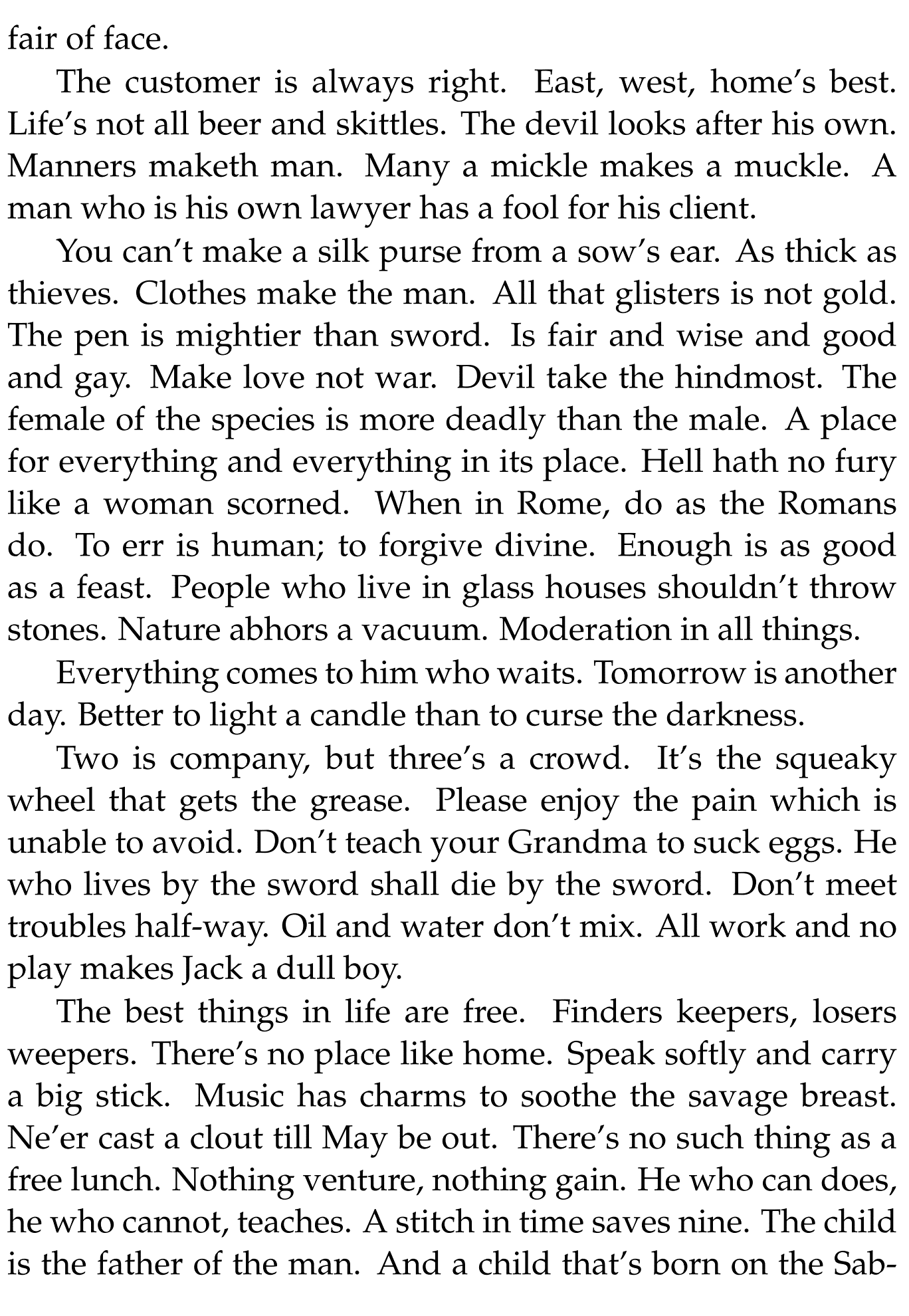附件3结果：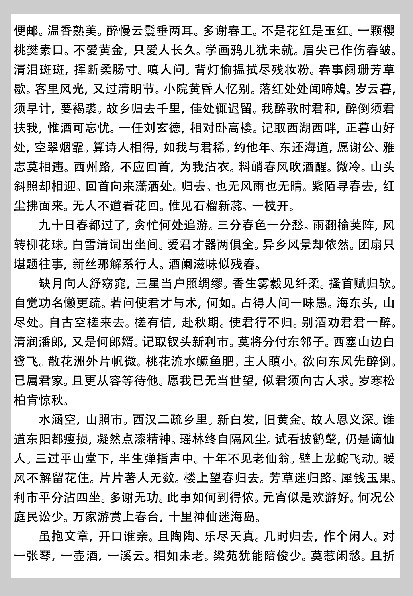附件4结果：附件5结果：
正面：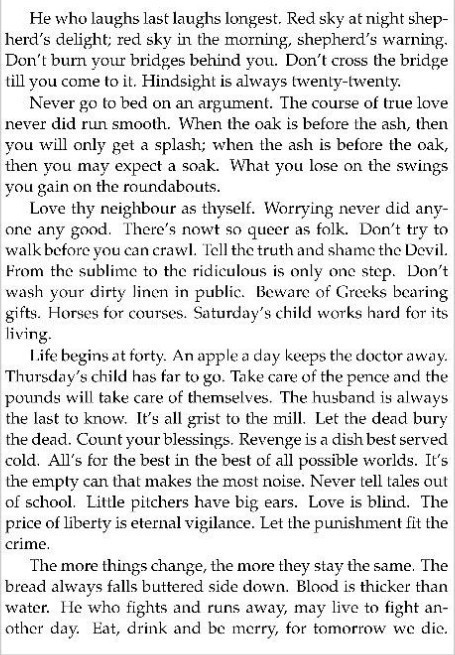反面：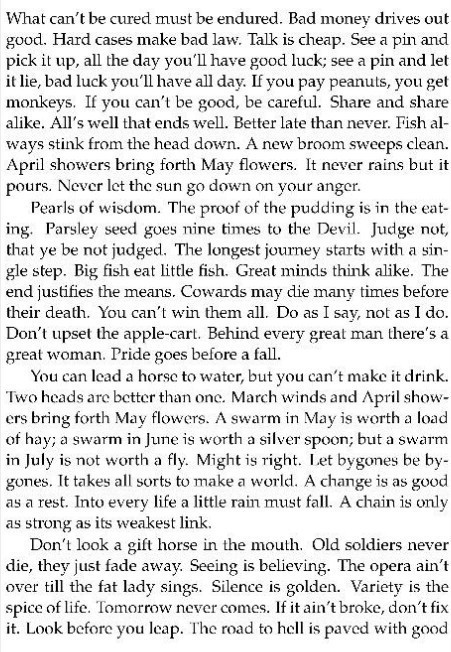展开全文• 通过MATLAB仿真实现规则图片与不规则图片的拼接复原工作。源自--华清
• 碎纸片拼接的讲解，内置有.m文件，有论文以及数据的处理和分析。
• 2013年数学建模B题相关资料,很好地实现了自动拼接
• 对于给定的来自同一页印刷文字文件的碎纸机破碎纸片（仅纵切），建立碎纸片拼接复原模型和算法，并针对附件1、附件2给出的中、英文各一页文件的碎片数据进行拼接复原。如果复原过程需要人工干预，请写出干预方式及...matlab训练
• 破碎文件的拼接在司法物证复原、历史文献修复以及军事情报获取等领域都有着重要的应用。...1. 对于给定的来自同一页印刷文字文件的碎纸机破碎纸片（仅纵切），建立碎纸片拼接复原模型和算法，并针对附件1、...